Скачать презентацию Lecture Outlines Chapter 17 Physics 3 rd Edition

• Количество слайдов: 85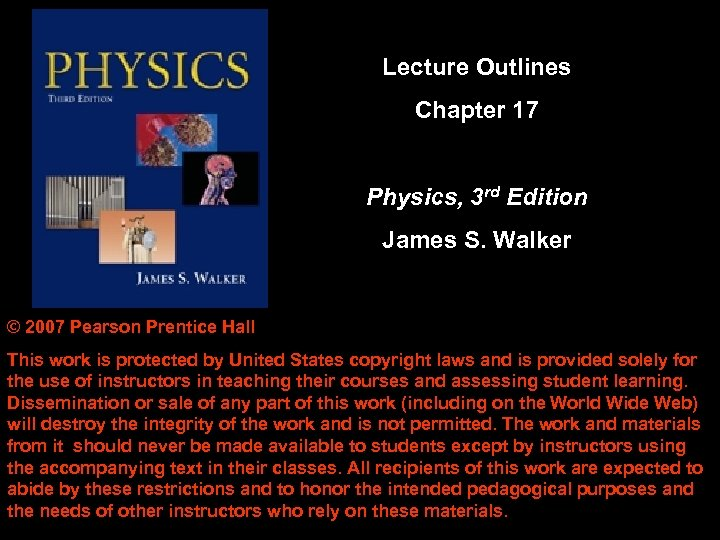Lecture Outlines Chapter 17 Physics, 3 rd Edition James S. Walker © 2007 Pearson Prentice Hall This work is protected by United States copyright laws and is provided solely for the use of instructors in teaching their courses and assessing student learning. Dissemination or sale of any part of this work (including on the World Wide Web) will destroy the integrity of the work and is not permitted. The work and materials from it should never be made available to students except by instructors using the accompanying text in their classes. All recipients of this work are expected to abide by these restrictions and to honor the intended pedagogical purposes and the needs of other instructors who rely on these materials.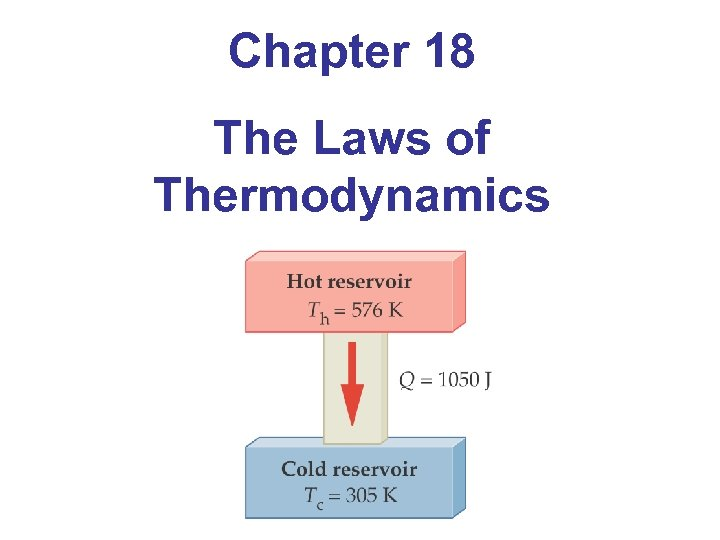Chapter 18 The Laws of Thermodynamics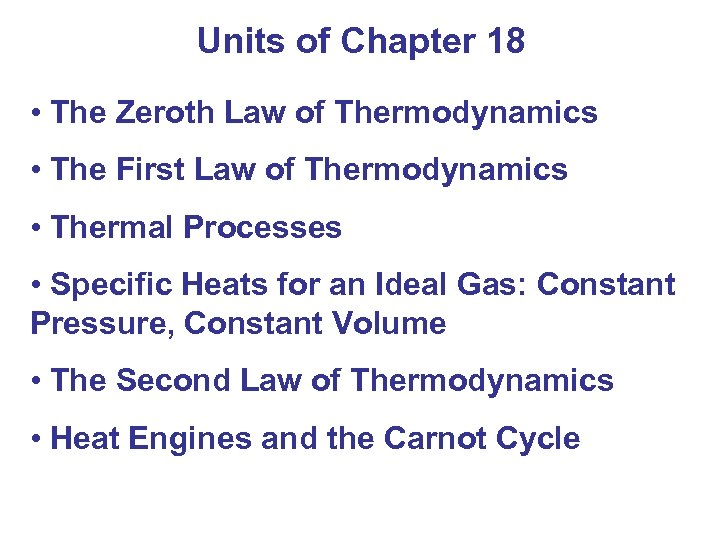Units of Chapter 18 • The Zeroth Law of Thermodynamics • The First Law of Thermodynamics • Thermal Processes • Specific Heats for an Ideal Gas: Constant Pressure, Constant Volume • The Second Law of Thermodynamics • Heat Engines and the Carnot Cycle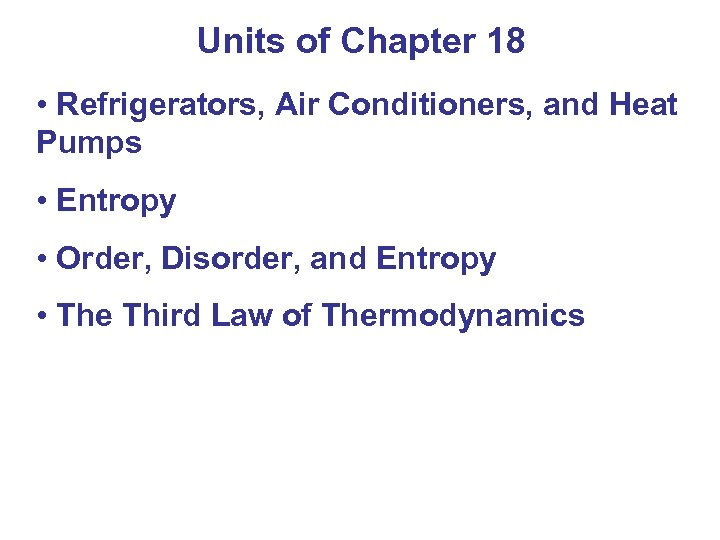Units of Chapter 18 • Refrigerators, Air Conditioners, and Heat Pumps • Entropy • Order, Disorder, and Entropy • The Third Law of Thermodynamics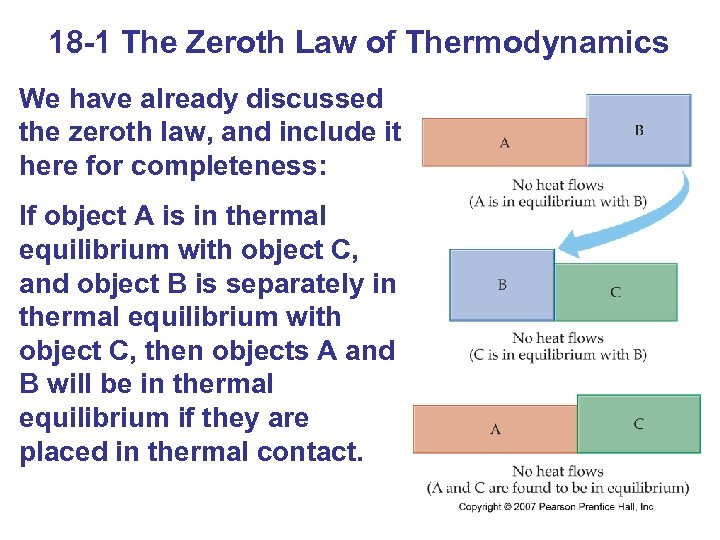18 -1 The Zeroth Law of Thermodynamics We have already discussed the zeroth law, and include it here for completeness: If object A is in thermal equilibrium with object C, and object B is separately in thermal equilibrium with object C, then objects A and B will be in thermal equilibrium if they are placed in thermal contact.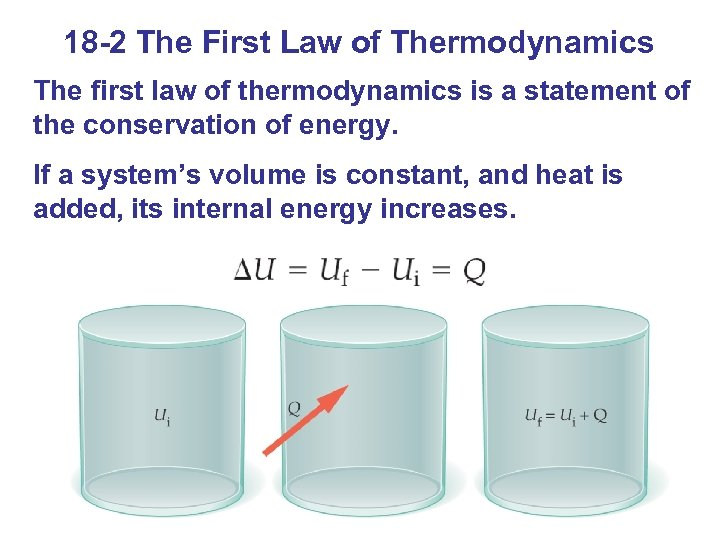18 -2 The First Law of Thermodynamics The first law of thermodynamics is a statement of the conservation of energy. If a system’s volume is constant, and heat is added, its internal energy increases.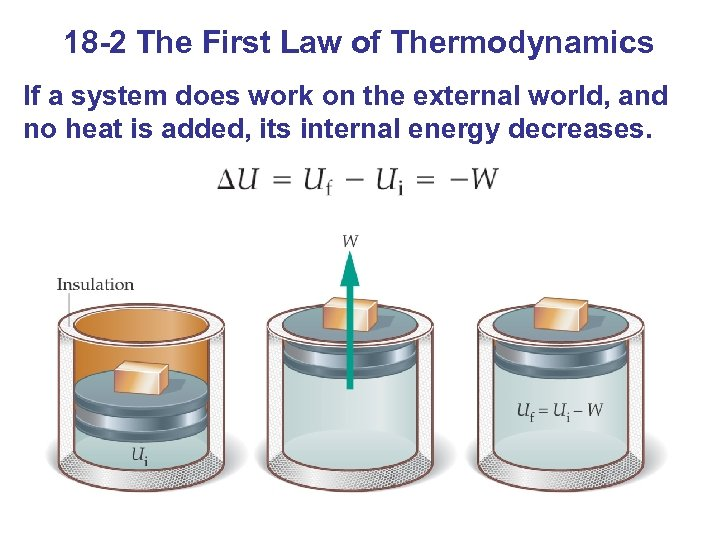18 -2 The First Law of Thermodynamics If a system does work on the external world, and no heat is added, its internal energy decreases.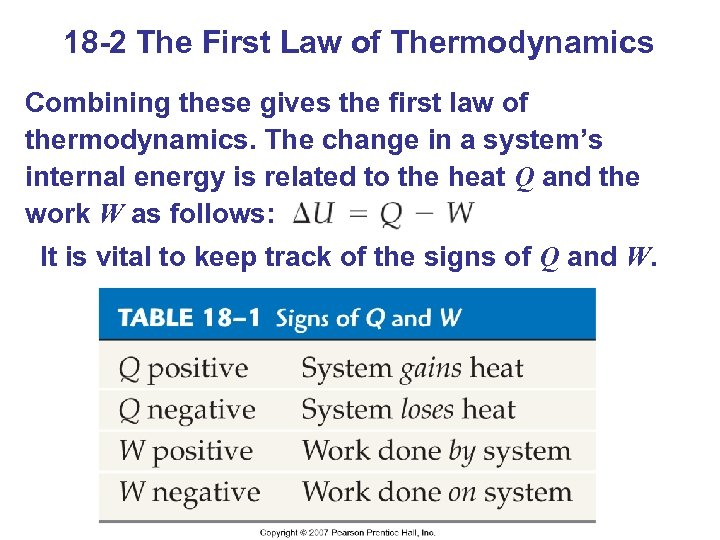18 -2 The First Law of Thermodynamics Combining these gives the first law of thermodynamics. The change in a system’s internal energy is related to the heat Q and the work W as follows: It is vital to keep track of the signs of Q and W.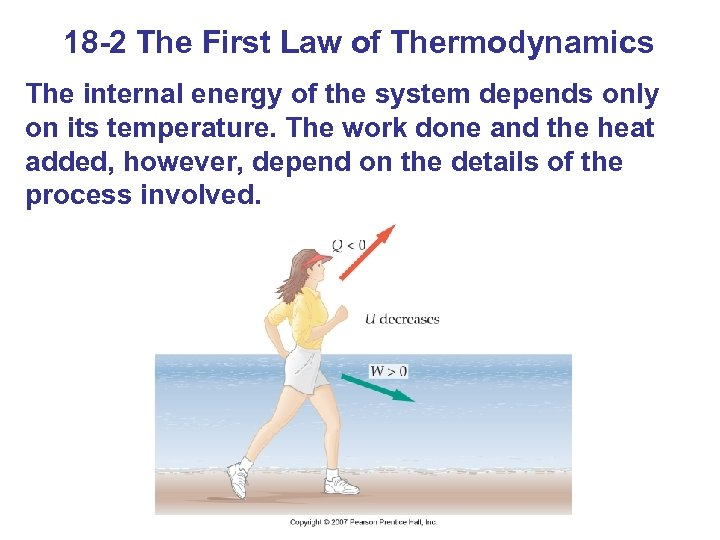18 -2 The First Law of Thermodynamics The internal energy of the system depends only on its temperature. The work done and the heat added, however, depend on the details of the process involved.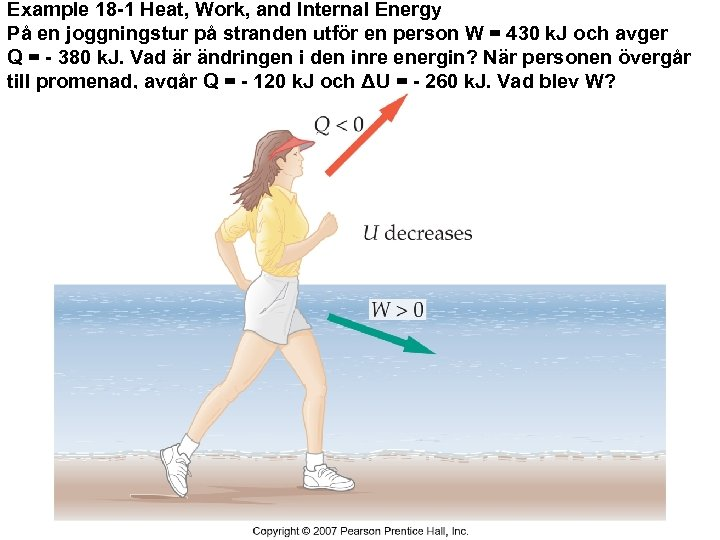Example 18 -1 Heat, Work, and Internal Energy På en joggningstur på stranden utför en person W = 430 k. J och avger Q = - 380 k. J. Vad är ändringen i den inre energin? När personen övergår till promenad, avgår Q = - 120 k. J och ΔU = - 260 k. J. Vad blev W?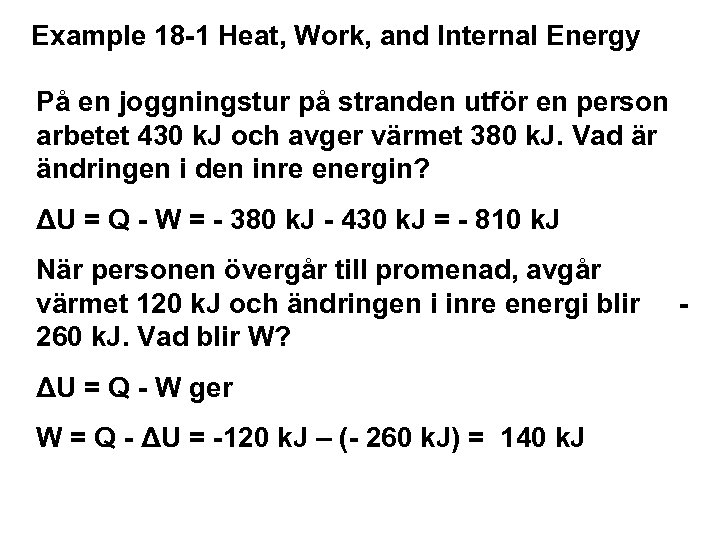Example 18 -1 Heat, Work, and Internal Energy På en joggningstur på stranden utför en person arbetet 430 k. J och avger värmet 380 k. J. Vad är ändringen i den inre energin? ΔU = Q - W = - 380 k. J - 430 k. J = - 810 k. J När personen övergår till promenad, avgår värmet 120 k. J och ändringen i inre energi blir 260 k. J. Vad blir W? ΔU = Q - W ger W = Q - ΔU = -120 k. J – (- 260 k. J) = 140 k. J -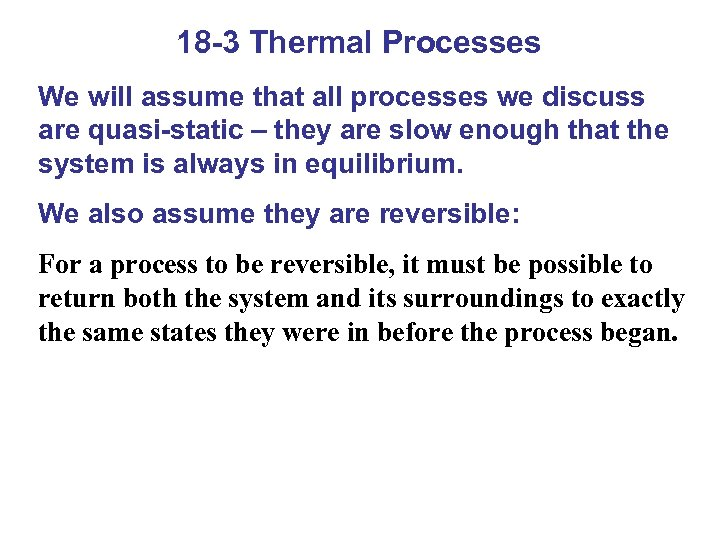18 -3 Thermal Processes We will assume that all processes we discuss are quasi-static – they are slow enough that the system is always in equilibrium. We also assume they are reversible: For a process to be reversible, it must be possible to return both the system and its surroundings to exactly the same states they were in before the process began.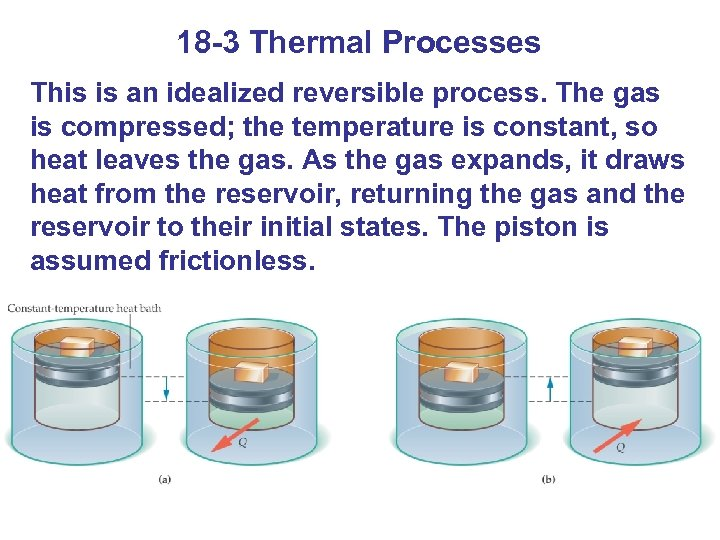18 -3 Thermal Processes This is an idealized reversible process. The gas is compressed; the temperature is constant, so heat leaves the gas. As the gas expands, it draws heat from the reservoir, returning the gas and the reservoir to their initial states. The piston is assumed frictionless.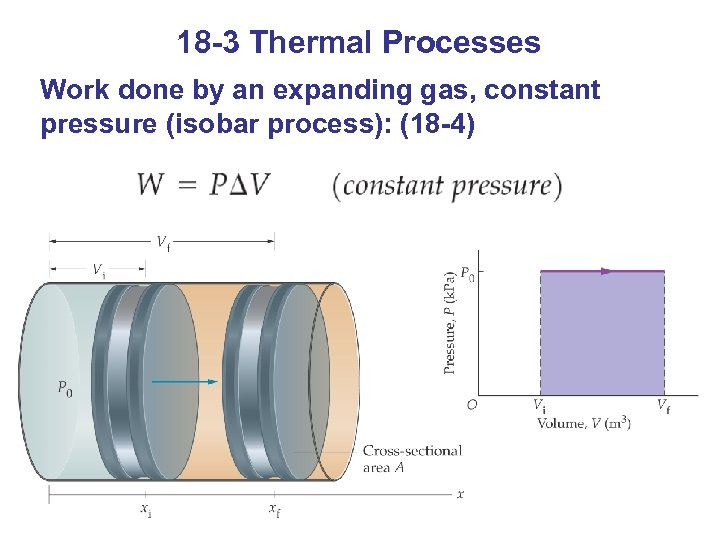18 -3 Thermal Processes Work done by an expanding gas, constant pressure (isobar process): (18 -4)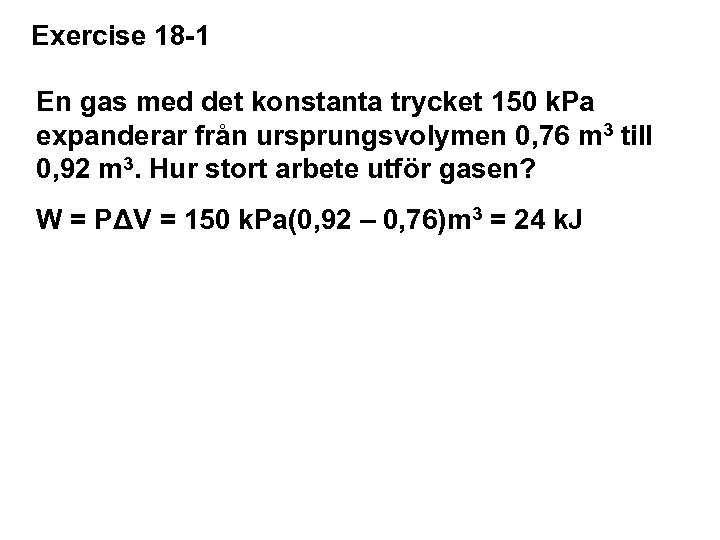Exercise 18 -1 En gas med det konstanta trycket 150 k. Pa expanderar från ursprungsvolymen 0, 76 m 3 till 0, 92 m 3. Hur stort arbete utför gasen? W = PΔV = 150 k. Pa(0, 92 – 0, 76)m 3 = 24 k. J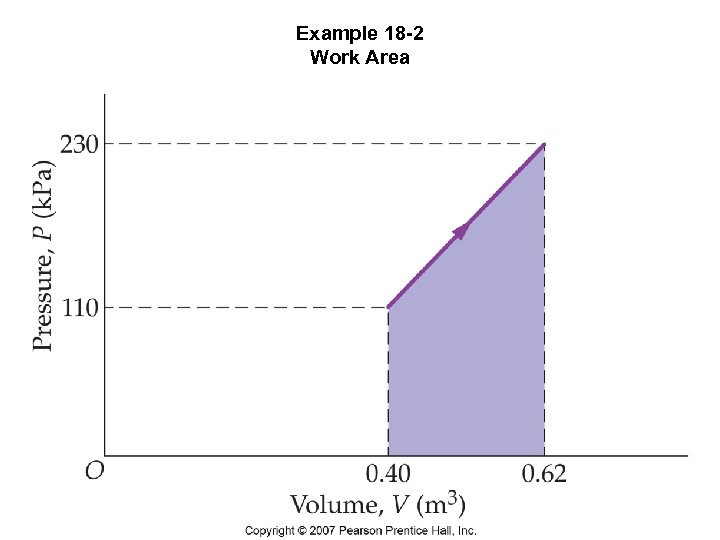Example 18 -2 Work Area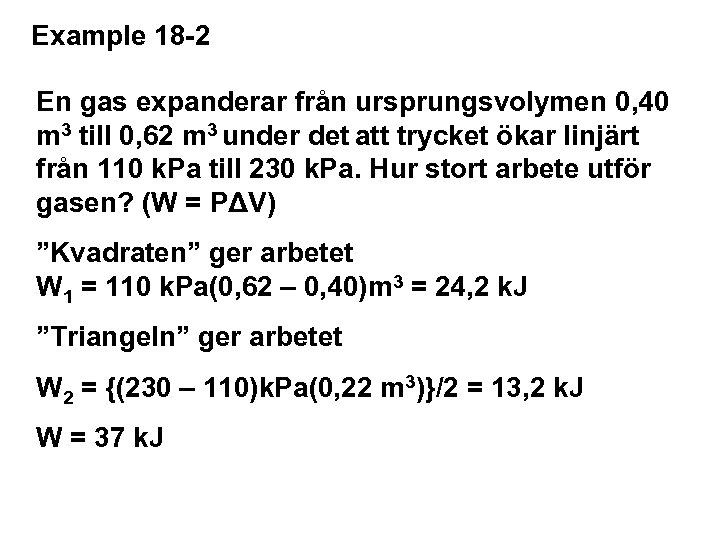Example 18 -2 En gas expanderar från ursprungsvolymen 0, 40 m 3 till 0, 62 m 3 under det att trycket ökar linjärt från 110 k. Pa till 230 k. Pa. Hur stort arbete utför gasen? (W = PΔV) ”Kvadraten” ger arbetet W 1 = 110 k. Pa(0, 62 – 0, 40)m 3 = 24, 2 k. J ”Triangeln” ger arbetet W 2 = {(230 – 110)k. Pa(0, 22 m 3)}/2 = 13, 2 k. J W = 37 k. J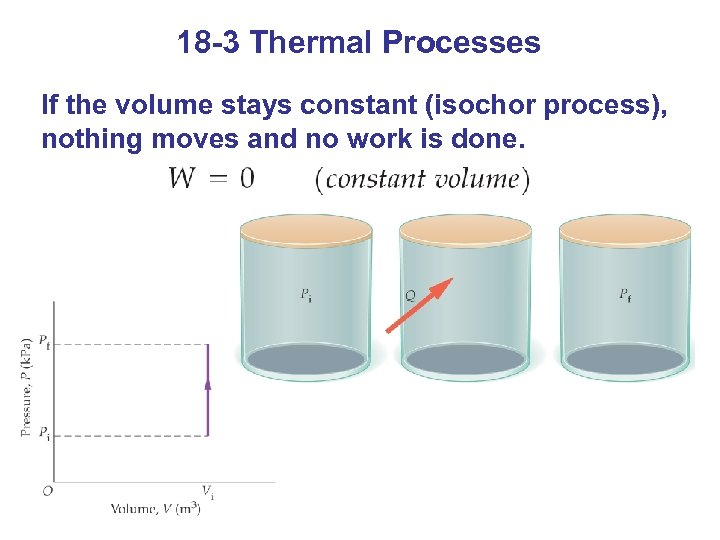18 -3 Thermal Processes If the volume stays constant (isochor process), nothing moves and no work is done.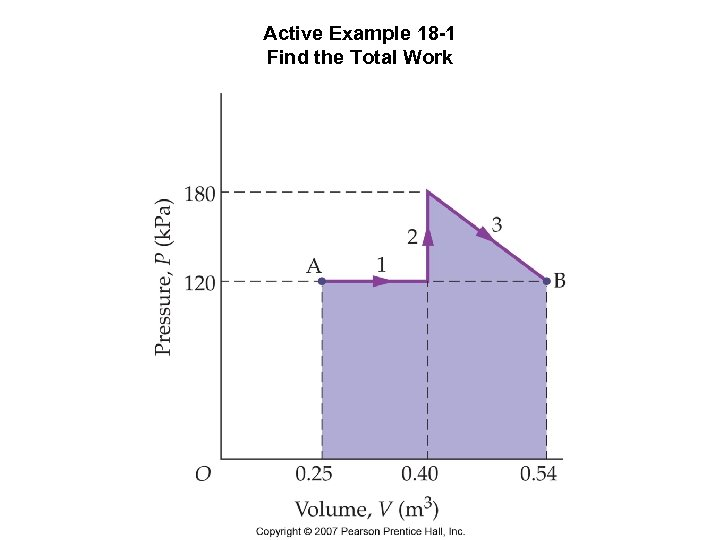Active Example 18 -1 Find the Total Work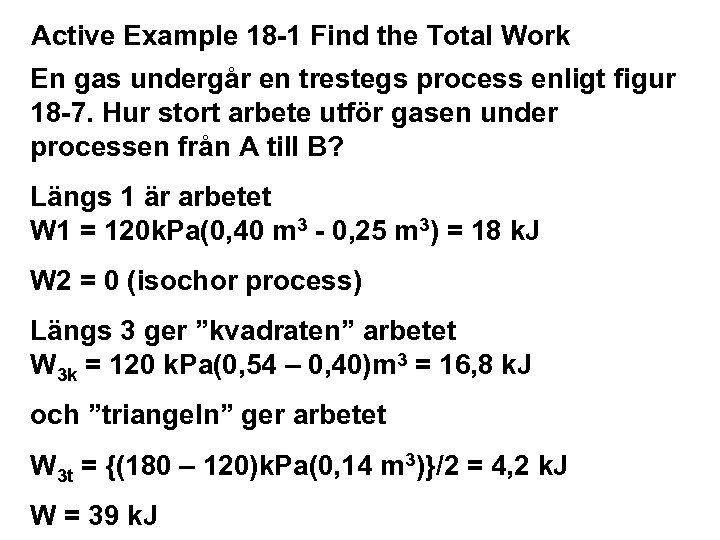Active Example 18 -1 Find the Total Work En gas undergår en trestegs process enligt figur 18 -7. Hur stort arbete utför gasen under processen från A till B? Längs 1 är arbetet W 1 = 120 k. Pa(0, 40 m 3 - 0, 25 m 3) = 18 k. J W 2 = 0 (isochor process) Längs 3 ger ”kvadraten” arbetet W 3 k = 120 k. Pa(0, 54 – 0, 40)m 3 = 16, 8 k. J och ”triangeln” ger arbetet W 3 t = {(180 – 120)k. Pa(0, 14 m 3)}/2 = 4, 2 k. J W = 39 k. J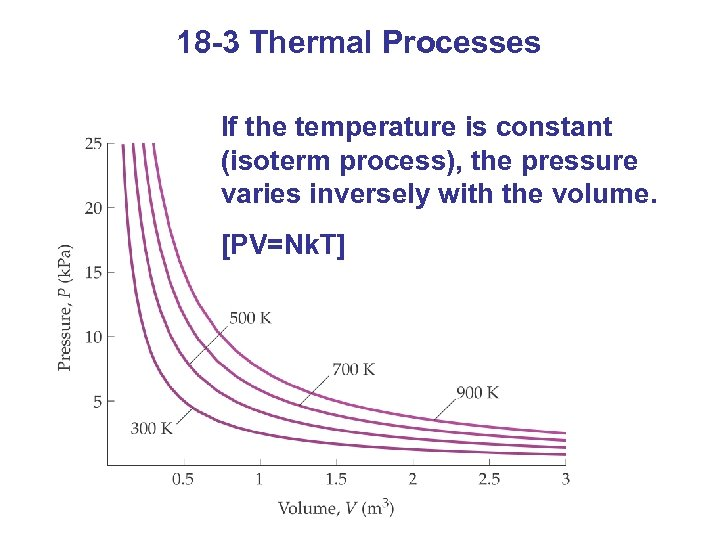18 -3 Thermal Processes If the temperature is constant (isoterm process), the pressure varies inversely with the volume. [PV=Nk. T]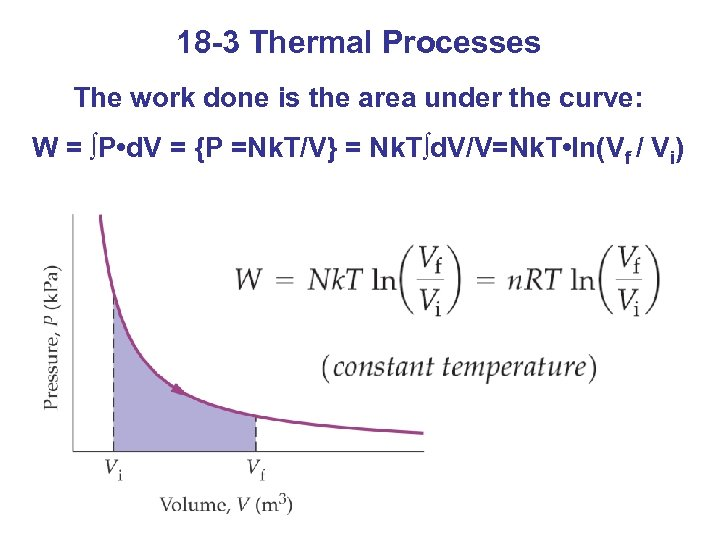18 -3 Thermal Processes The work done is the area under the curve: W = ∫P • d. V = {P =Nk. T/V} = Nk. T∫d. V/V=Nk. T • ln(Vf / Vi)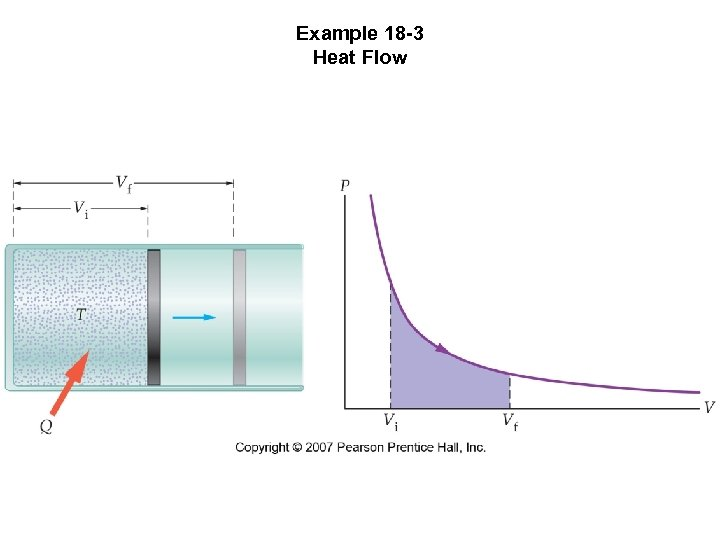Example 18 -3 Heat Flow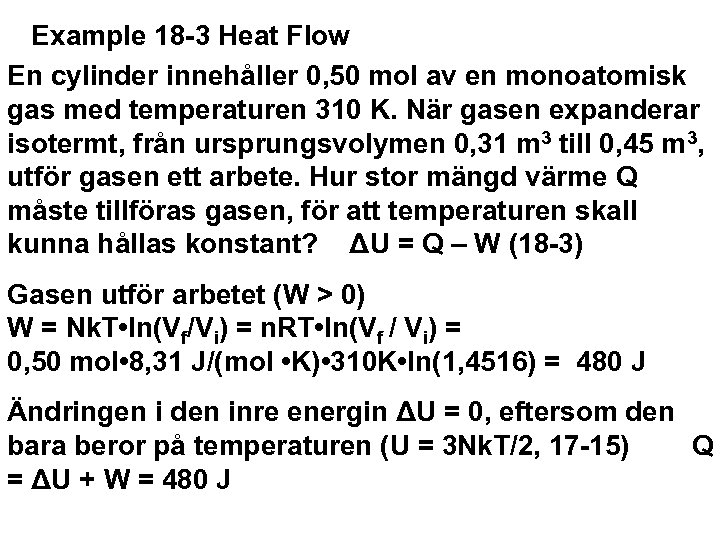Example 18 -3 Heat Flow En cylinder innehåller 0, 50 mol av en monoatomisk gas med temperaturen 310 K. När gasen expanderar isotermt, från ursprungsvolymen 0, 31 m 3 till 0, 45 m 3, utför gasen ett arbete. Hur stor mängd värme Q måste tillföras gasen, för att temperaturen skall kunna hållas konstant? ΔU = Q – W (18 -3) Gasen utför arbetet (W > 0) W = Nk. T • ln(Vf/Vi) = n. RT • ln(Vf / Vi) = 0, 50 mol • 8, 31 J/(mol • K) • 310 K • ln(1, 4516) = 480 J Ändringen i den inre energin ΔU = 0, eftersom den bara beror på temperaturen (U = 3 Nk. T/2, 17 -15) Q = ΔU + W = 480 J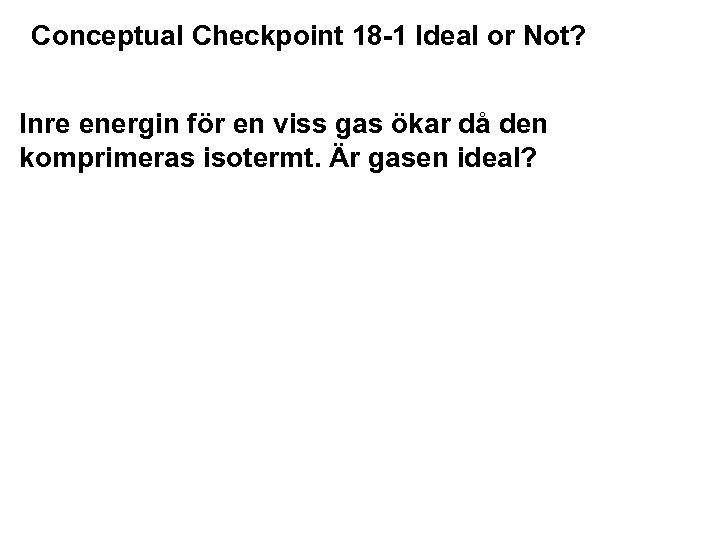Conceptual Checkpoint 18 -1 Ideal or Not? Inre energin för en viss gas ökar då den komprimeras isotermt. Är gasen ideal?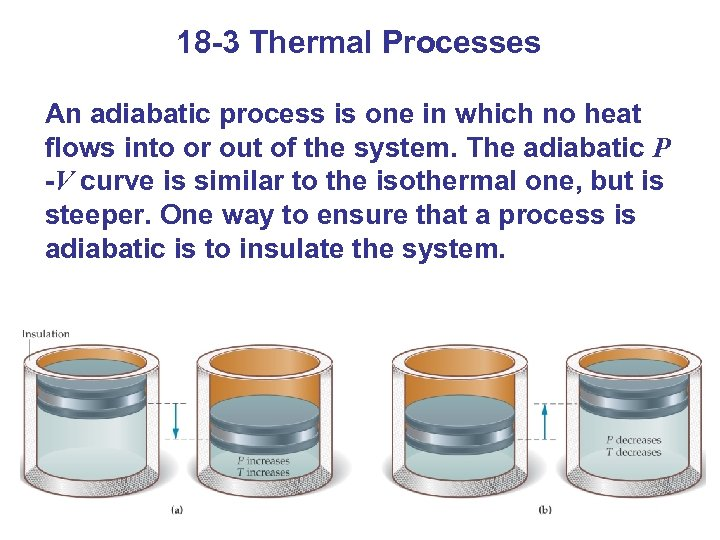18 -3 Thermal Processes An adiabatic process is one in which no heat flows into or out of the system. The adiabatic P -V curve is similar to the isothermal one, but is steeper. One way to ensure that a process is adiabatic is to insulate the system.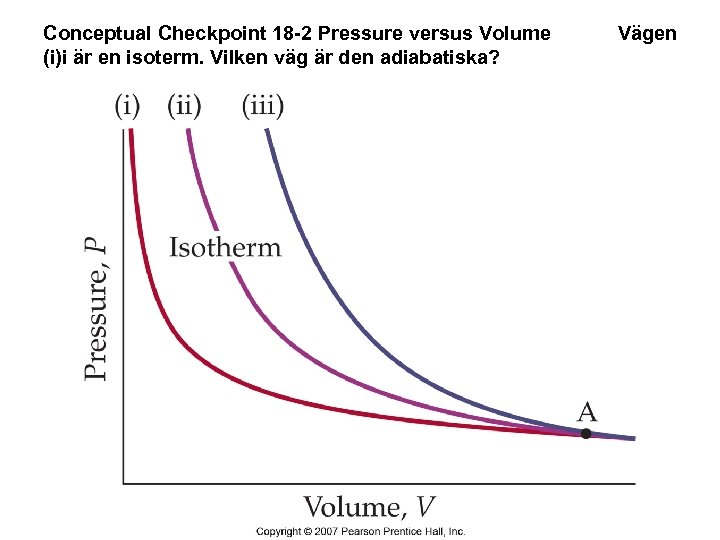Conceptual Checkpoint 18 -2 Pressure versus Volume (i)i är en isoterm. Vilken väg är den adiabatiska? Vägen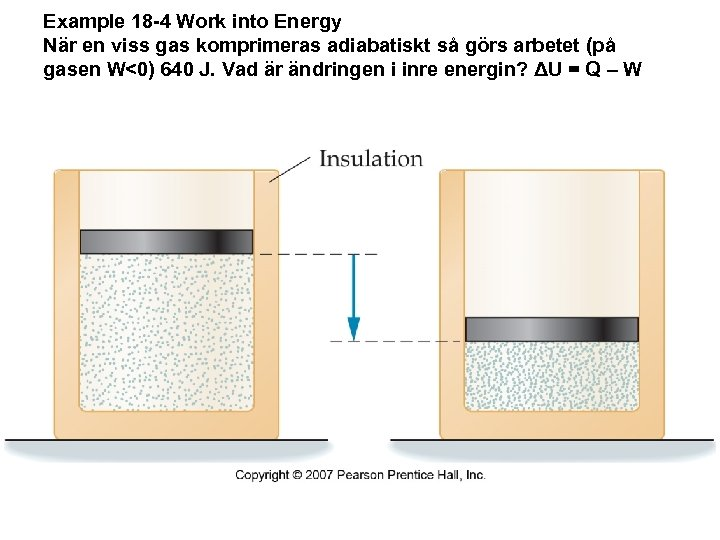Example 18 -4 Work into Energy När en viss gas komprimeras adiabatiskt så görs arbetet (på gasen W<0) 640 J. Vad är ändringen i inre energin? ΔU = Q – W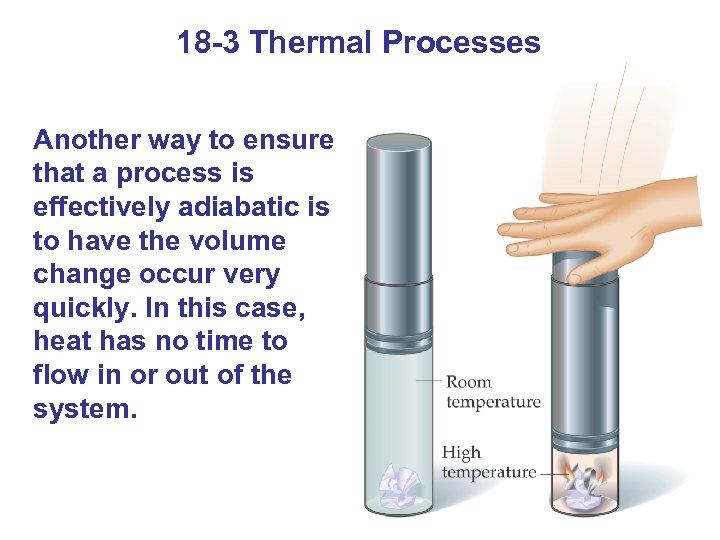18 -3 Thermal Processes Another way to ensure that a process is effectively adiabatic is to have the volume change occur very quickly. In this case, heat has no time to flow in or out of the system.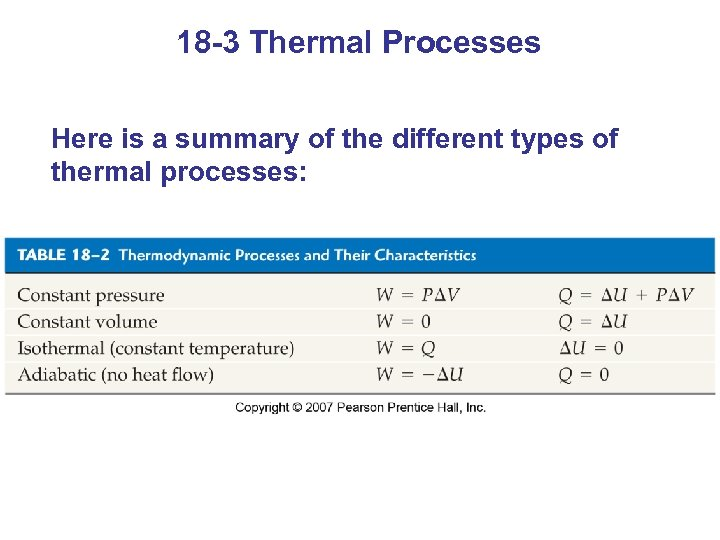18 -3 Thermal Processes Here is a summary of the different types of thermal processes: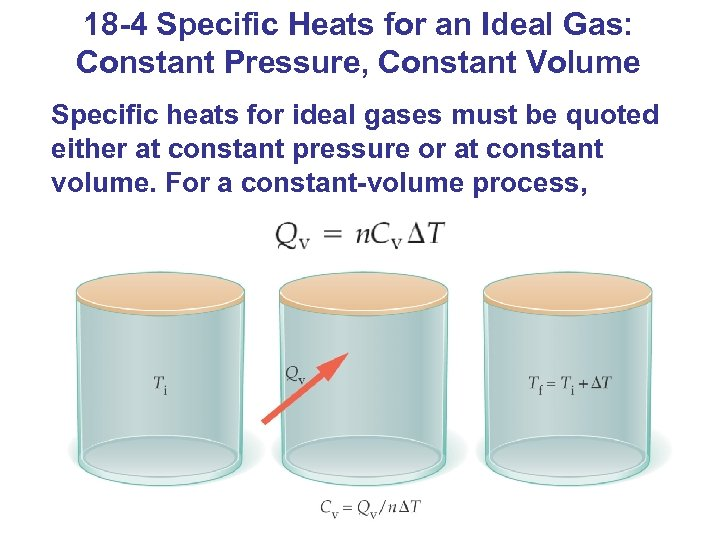18 -4 Specific Heats for an Ideal Gas: Constant Pressure, Constant Volume Specific heats for ideal gases must be quoted either at constant pressure or at constant volume. For a constant-volume process,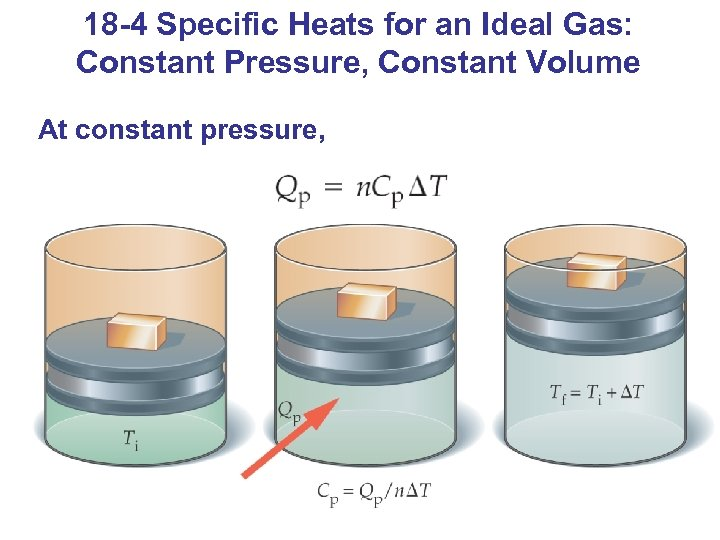18 -4 Specific Heats for an Ideal Gas: Constant Pressure, Constant Volume At constant pressure,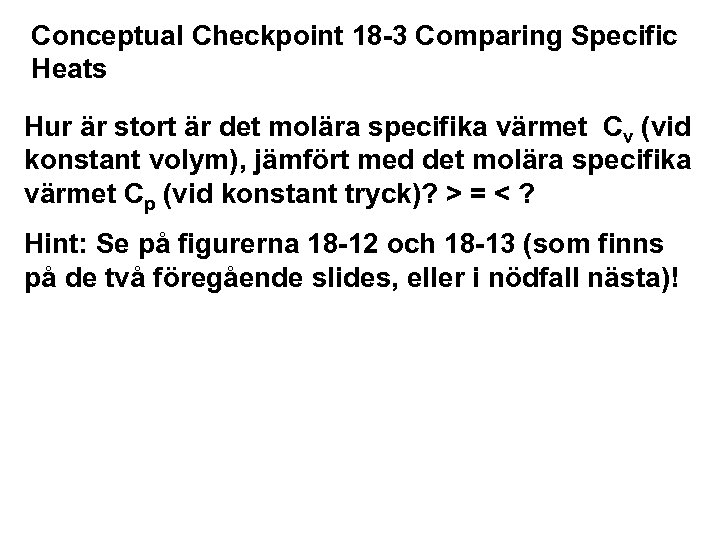Conceptual Checkpoint 18 -3 Comparing Specific Heats Hur är stort är det molära specifika värmet Cv (vid konstant volym), jämfört med det molära specifika värmet Cp (vid konstant tryck)? > = < ? Hint: Se på figurerna 18 -12 och 18 -13 (som finns på de två föregående slides, eller i nödfall nästa)!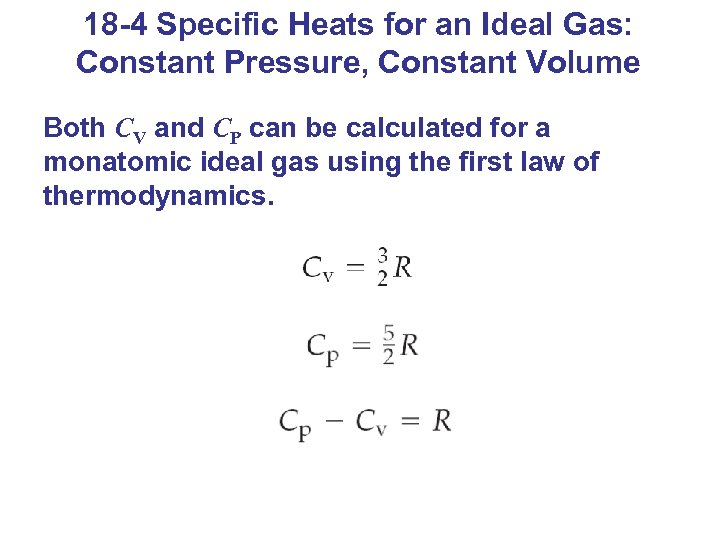18 -4 Specific Heats for an Ideal Gas: Constant Pressure, Constant Volume Both CV and CP can be calculated for a monatomic ideal gas using the first law of thermodynamics.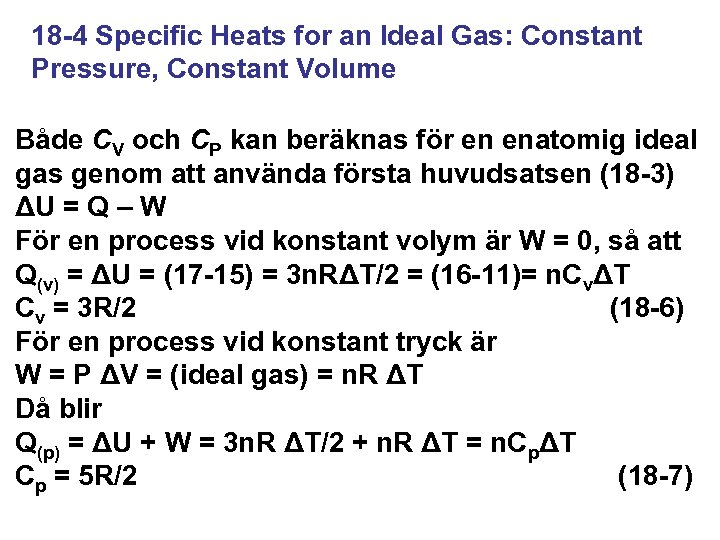18 -4 Specific Heats for an Ideal Gas: Constant Pressure, Constant Volume Både CV och CP kan beräknas för en enatomig ideal gas genom att använda första huvudsatsen (18 -3) ΔU = Q – W För en process vid konstant volym är W = 0, så att Q(v) = ΔU = (17 -15) = 3 n. RΔT/2 = (16 -11)= n. CvΔT Cv = 3 R/2 (18 -6) För en process vid konstant tryck är W = P ΔV = (ideal gas) = n. R ΔT Då blir Q(p) = ΔU + W = 3 n. R ΔT/2 + n. R ΔT = n. CpΔT Cp = 5 R/2 (18 -7)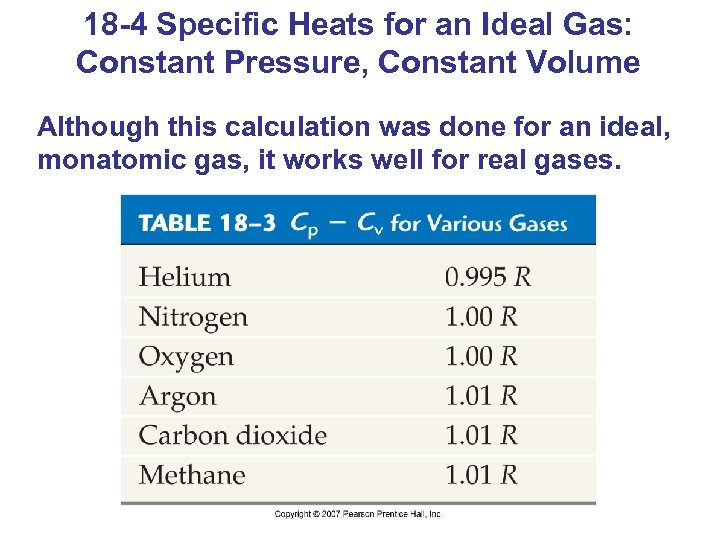18 -4 Specific Heats for an Ideal Gas: Constant Pressure, Constant Volume Although this calculation was done for an ideal, monatomic gas, it works well for real gases.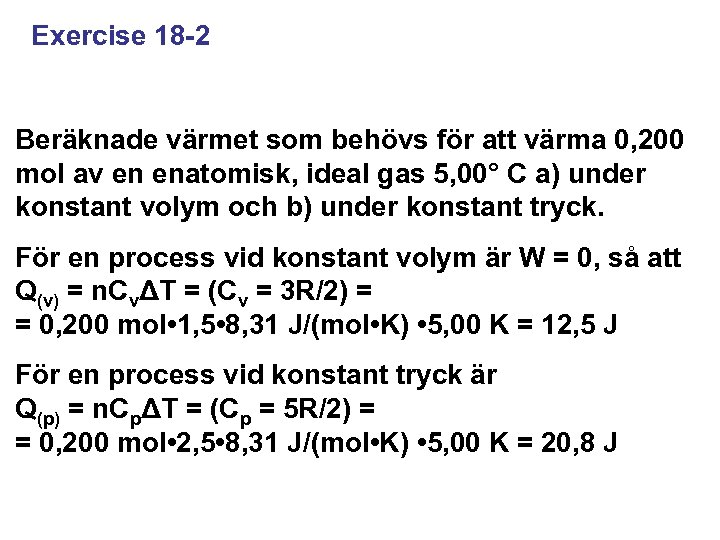Exercise 18 -2 Beräknade värmet som behövs för att värma 0, 200 mol av en enatomisk, ideal gas 5, 00° C a) under konstant volym och b) under konstant tryck. För en process vid konstant volym är W = 0, så att Q(v) = n. CvΔT = (Cv = 3 R/2) = = 0, 200 mol • 1, 5 • 8, 31 J/(mol • K) • 5, 00 K = 12, 5 J För en process vid konstant tryck är Q(p) = n. CpΔT = (Cp = 5 R/2) = = 0, 200 mol • 2, 5 • 8, 31 J/(mol • K) • 5, 00 K = 20, 8 J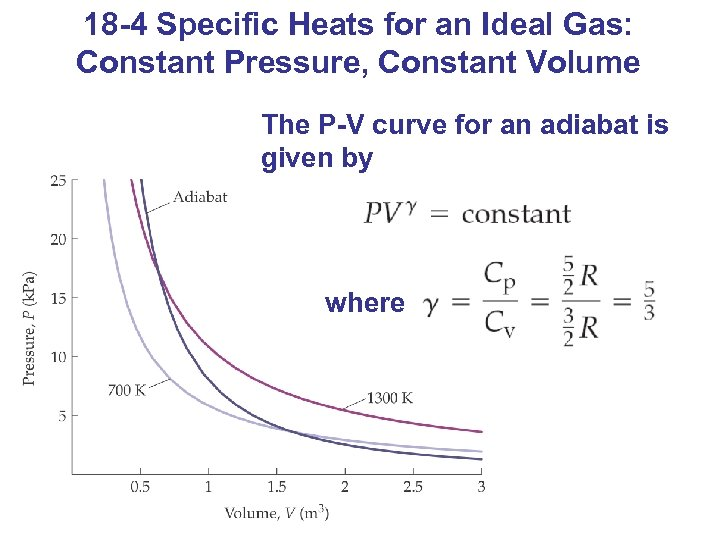18 -4 Specific Heats for an Ideal Gas: Constant Pressure, Constant Volume The P-V curve for an adiabat is given by where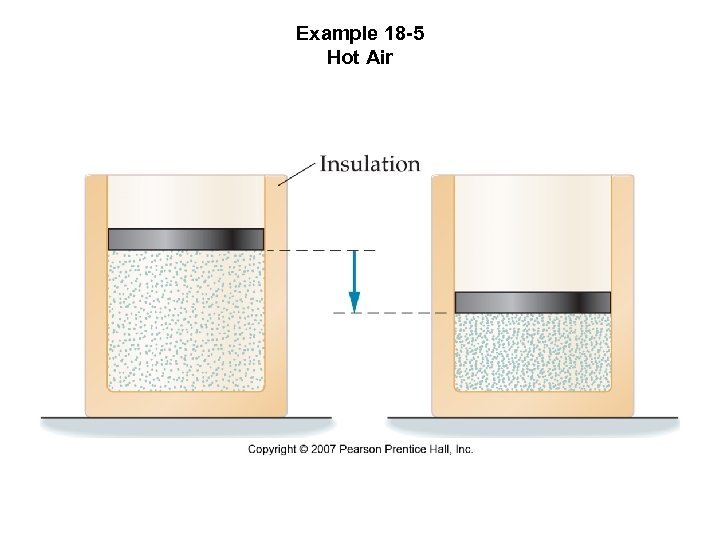Example 18 -5 Hot Air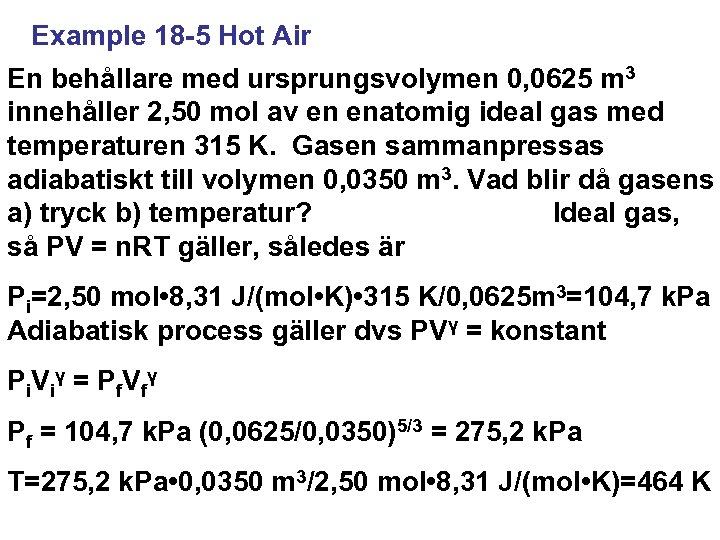Example 18 -5 Hot Air En behållare med ursprungsvolymen 0, 0625 m 3 innehåller 2, 50 mol av en enatomig ideal gas med temperaturen 315 K. Gasen sammanpressas adiabatiskt till volymen 0, 0350 m 3. Vad blir då gasens a) tryck b) temperatur? Ideal gas, så PV = n. RT gäller, således är Pi=2, 50 mol • 8, 31 J/(mol • K) • 315 K/0, 0625 m 3=104, 7 k. Pa Adiabatisk process gäller dvs PVγ = konstant P i V i γ = P f. V fγ Pf = 104, 7 k. Pa (0, 0625/0, 0350)5/3 = 275, 2 k. Pa T=275, 2 k. Pa • 0, 0350 m 3/2, 50 mol • 8, 31 J/(mol • K)=464 K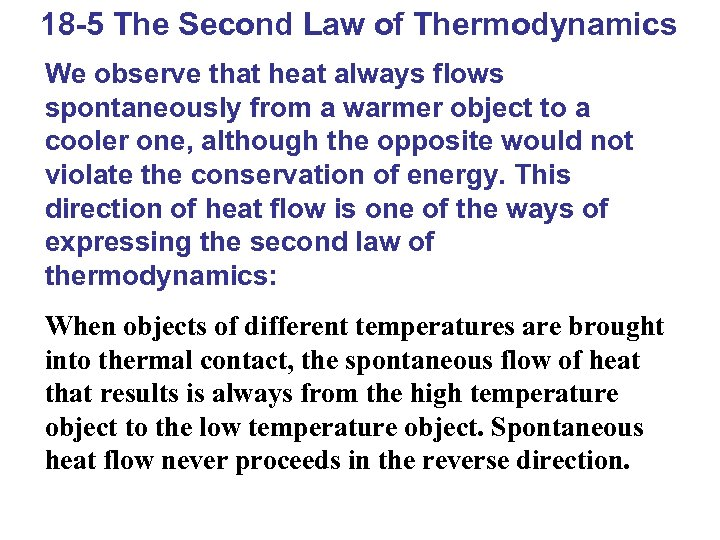18 -5 The Second Law of Thermodynamics We observe that heat always flows spontaneously from a warmer object to a cooler one, although the opposite would not violate the conservation of energy. This direction of heat flow is one of the ways of expressing the second law of thermodynamics: When objects of different temperatures are brought into thermal contact, the spontaneous flow of heat that results is always from the high temperature object to the low temperature object. Spontaneous heat flow never proceeds in the reverse direction.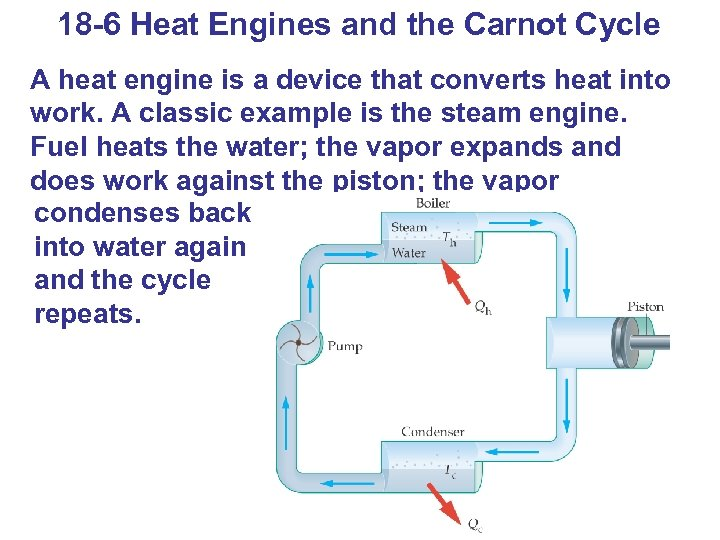18 -6 Heat Engines and the Carnot Cycle A heat engine is a device that converts heat into work. A classic example is the steam engine. Fuel heats the water; the vapor expands and does work against the piston; the vapor condenses back into water again and the cycle repeats.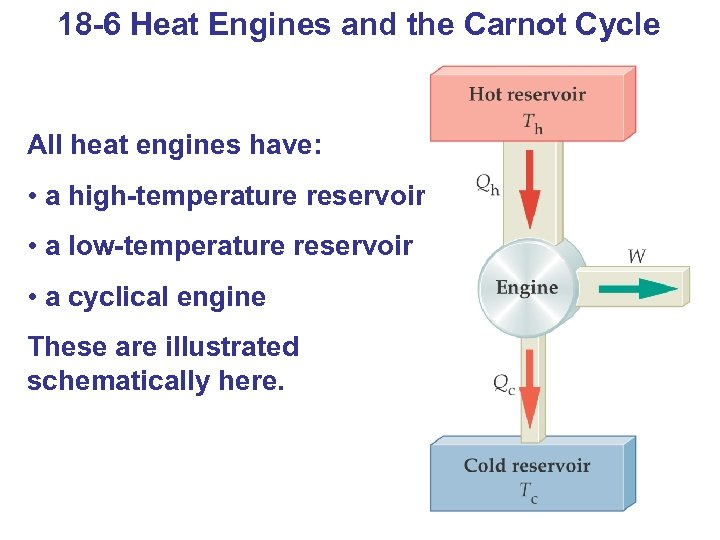18 -6 Heat Engines and the Carnot Cycle All heat engines have: • a high-temperature reservoir • a low-temperature reservoir • a cyclical engine These are illustrated schematically here.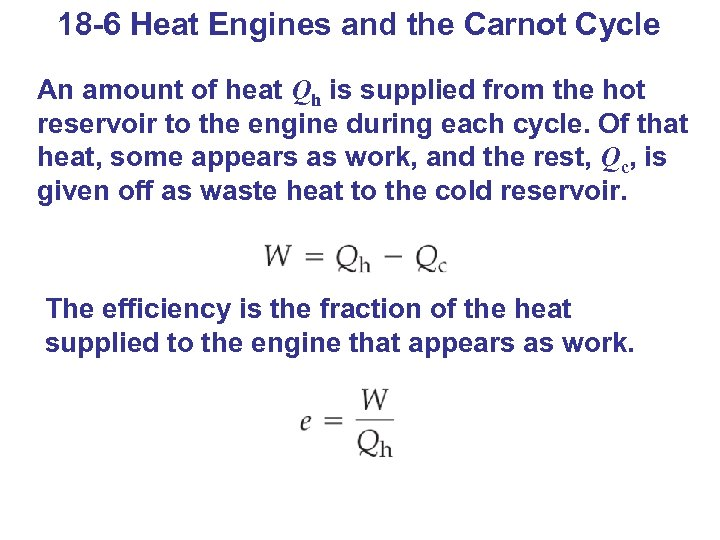18 -6 Heat Engines and the Carnot Cycle An amount of heat Qh is supplied from the hot reservoir to the engine during each cycle. Of that heat, some appears as work, and the rest, Qc, is given off as waste heat to the cold reservoir. The efficiency is the fraction of the heat supplied to the engine that appears as work.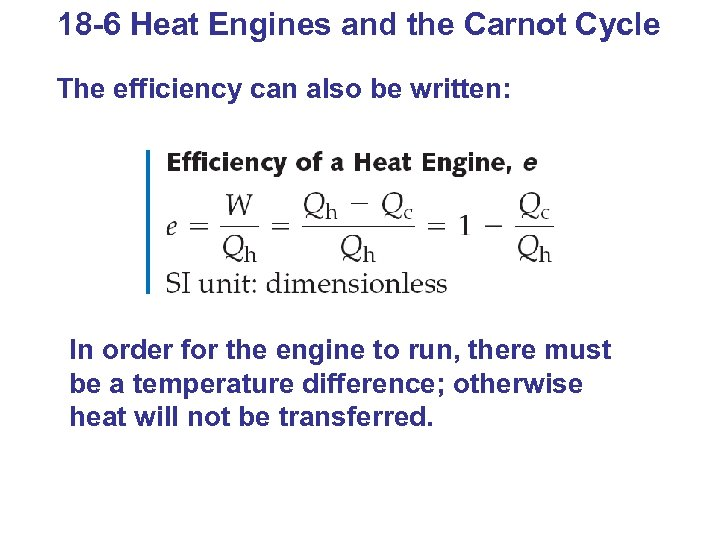18 -6 Heat Engines and the Carnot Cycle The efficiency can also be written: In order for the engine to run, there must be a temperature difference; otherwise heat will not be transferred.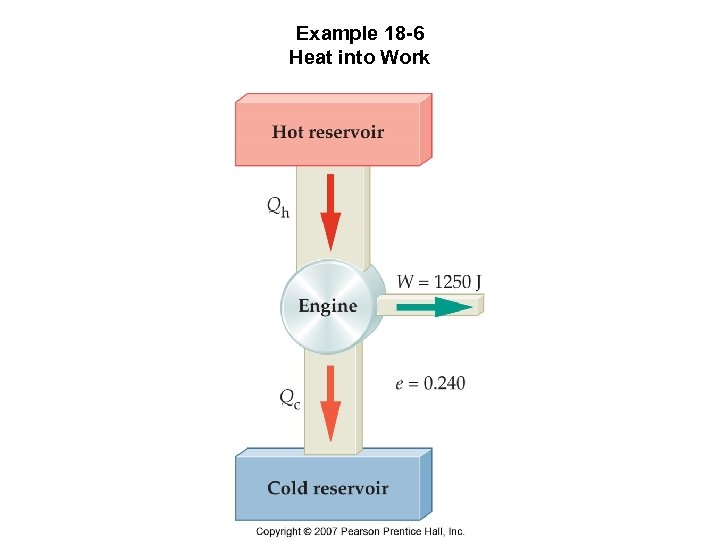Example 18 -6 Heat into Work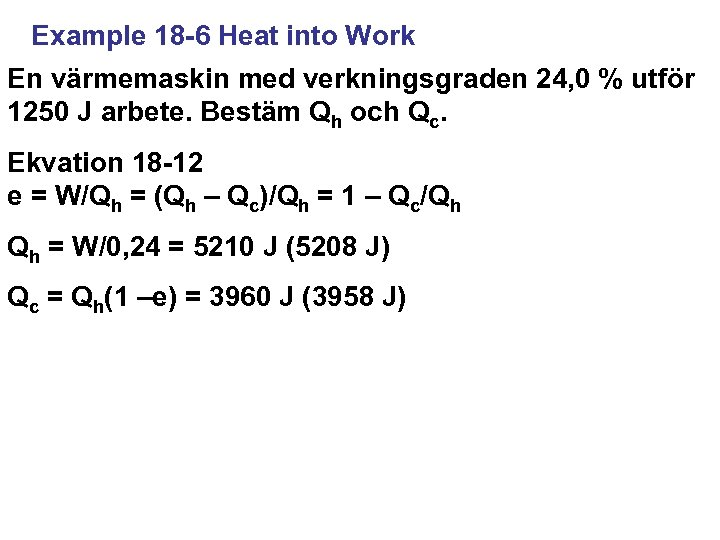Example 18 -6 Heat into Work En värmemaskin med verkningsgraden 24, 0 % utför 1250 J arbete. Bestäm Qh och Qc. Ekvation 18 -12 e = W/Qh = (Qh – Qc)/Qh = 1 – Qc/Qh Qh = W/0, 24 = 5210 J (5208 J) Qc = Qh(1 –e) = 3960 J (3958 J)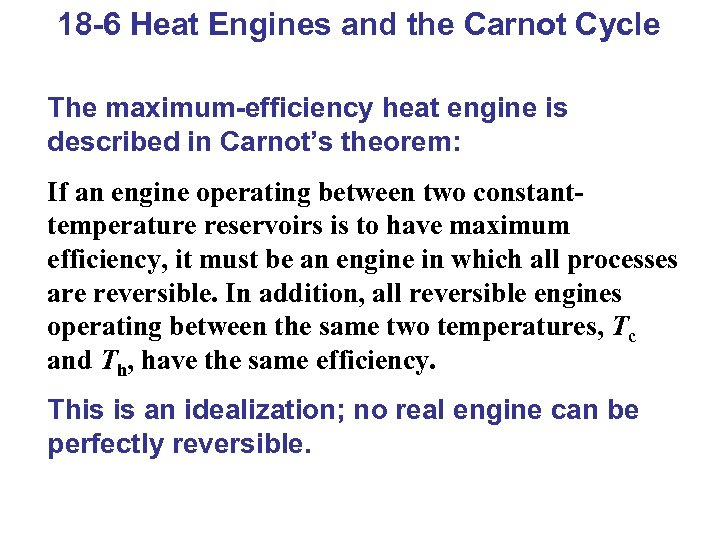18 -6 Heat Engines and the Carnot Cycle The maximum-efficiency heat engine is described in Carnot’s theorem: If an engine operating between two constanttemperature reservoirs is to have maximum efficiency, it must be an engine in which all processes are reversible. In addition, all reversible engines operating between the same two temperatures, Tc and Th, have the same efficiency. This is an idealization; no real engine can be perfectly reversible.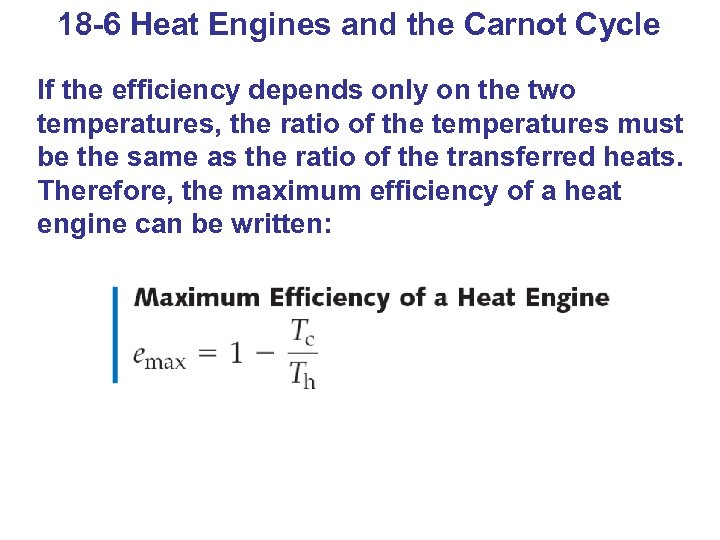18 -6 Heat Engines and the Carnot Cycle If the efficiency depends only on the two temperatures, the ratio of the temperatures must be the same as the ratio of the transferred heats. Therefore, the maximum efficiency of a heat engine can be written: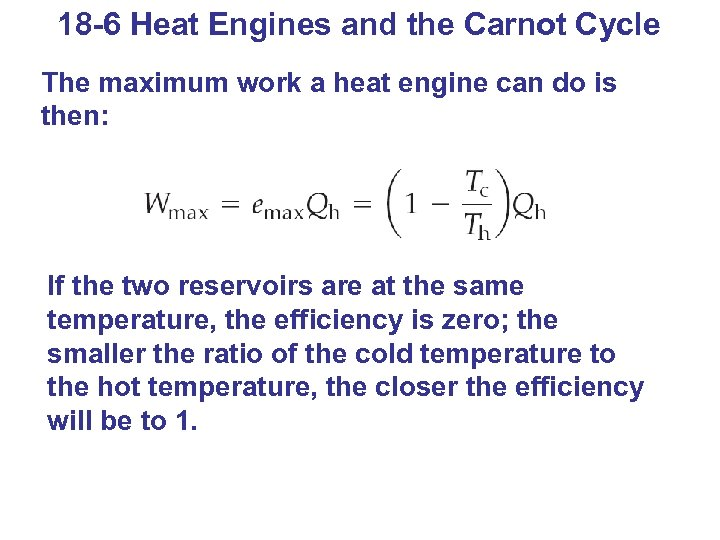18 -6 Heat Engines and the Carnot Cycle The maximum work a heat engine can do is then: If the two reservoirs are at the same temperature, the efficiency is zero; the smaller the ratio of the cold temperature to the hot temperature, the closer the efficiency will be to 1.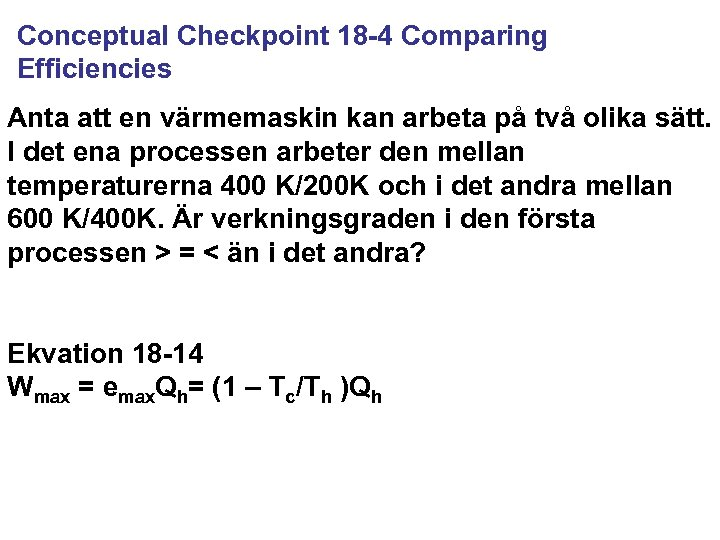Conceptual Checkpoint 18 -4 Comparing Efficiencies Anta att en värmemaskin kan arbeta på två olika sätt. I det ena processen arbeter den mellan temperaturerna 400 K/200 K och i det andra mellan 600 K/400 K. Är verkningsgraden i den första processen > = < än i det andra? Ekvation 18 -14 Wmax = emax. Qh= (1 – Tc/Th )Qh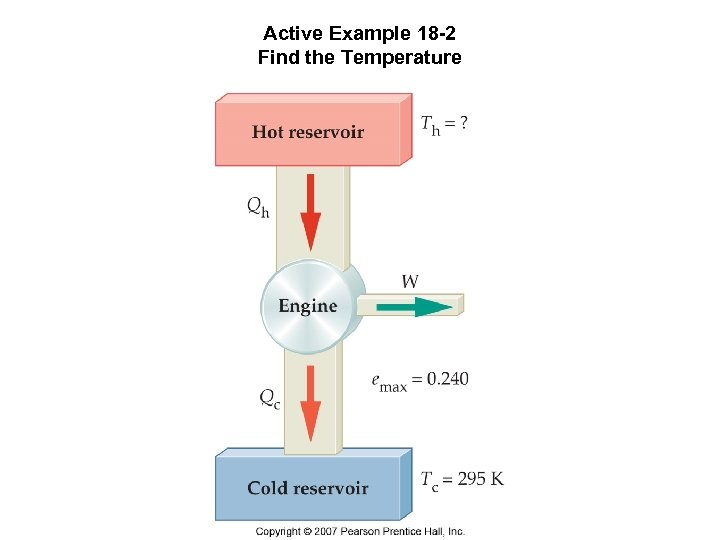Active Example 18 -2 Find the Temperature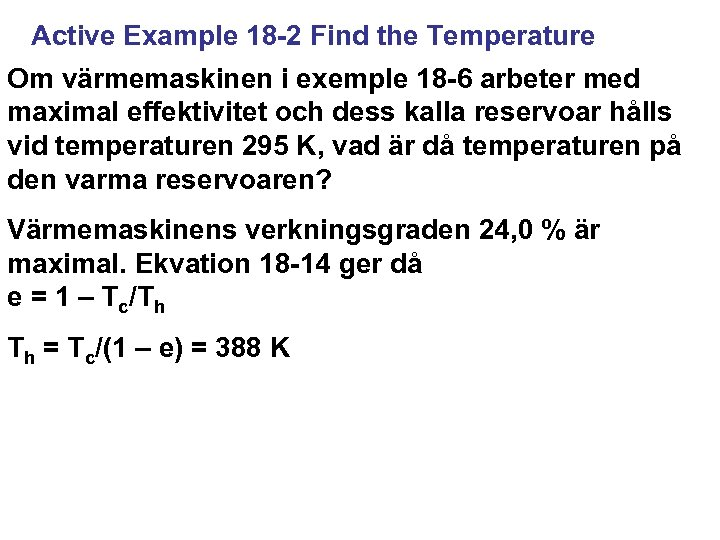Active Example 18 -2 Find the Temperature Om värmemaskinen i exemple 18 -6 arbeter med maximal effektivitet och dess kalla reservoar hålls vid temperaturen 295 K, vad är då temperaturen på den varma reservoaren? Värmemaskinens verkningsgraden 24, 0 % är maximal. Ekvation 18 -14 ger då e = 1 – Tc/Th Th = Tc/(1 – e) = 388 K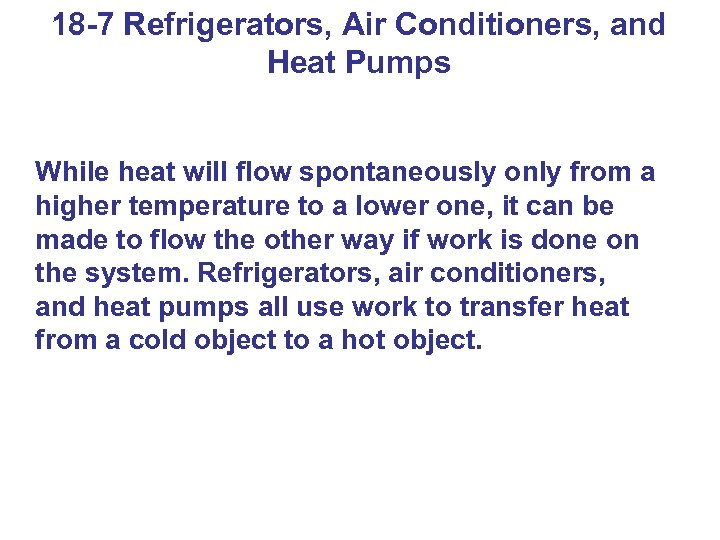18 -7 Refrigerators, Air Conditioners, and Heat Pumps While heat will flow spontaneously only from a higher temperature to a lower one, it can be made to flow the other way if work is done on the system. Refrigerators, air conditioners, and heat pumps all use work to transfer heat from a cold object to a hot object.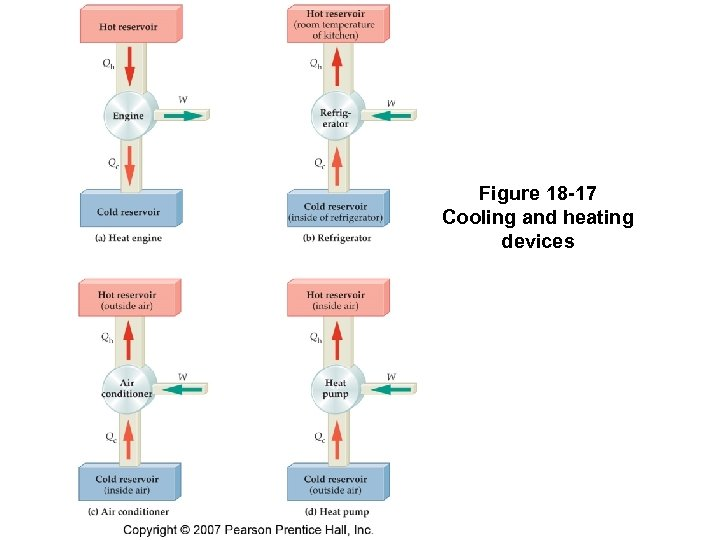Figure 18 -17 Cooling and heating devices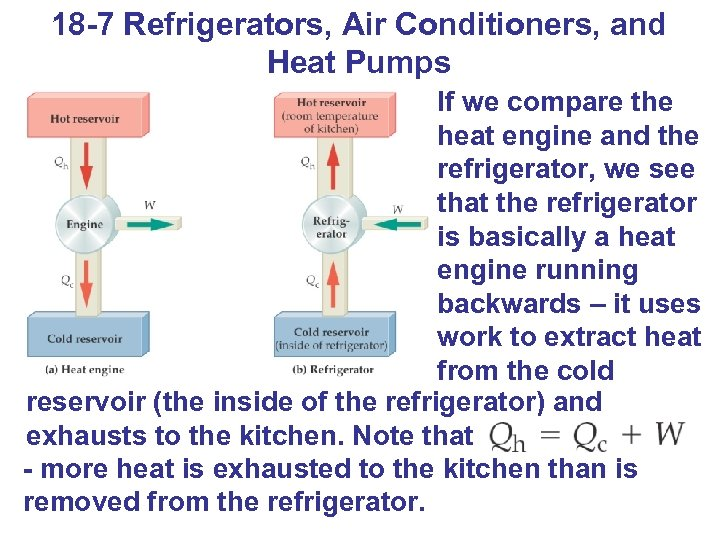18 -7 Refrigerators, Air Conditioners, and Heat Pumps If we compare the heat engine and the refrigerator, we see that the refrigerator is basically a heat engine running backwards – it uses work to extract heat from the cold reservoir (the inside of the refrigerator) and exhausts to the kitchen. Note that - more heat is exhausted to the kitchen than is removed from the refrigerator.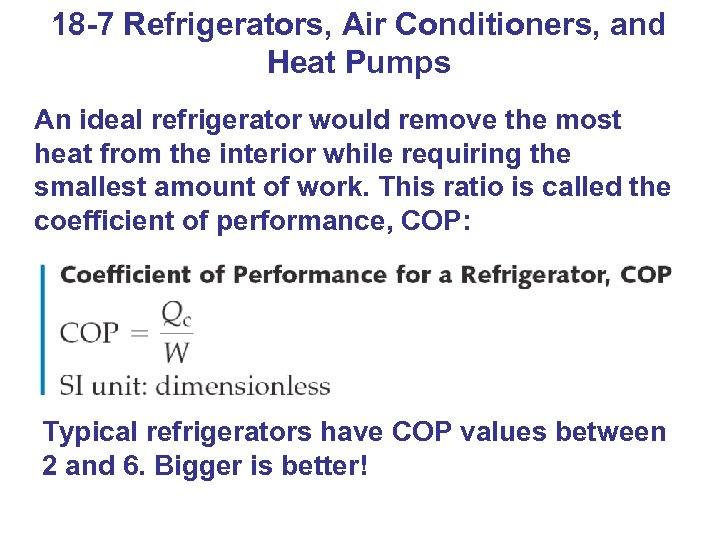18 -7 Refrigerators, Air Conditioners, and Heat Pumps An ideal refrigerator would remove the most heat from the interior while requiring the smallest amount of work. This ratio is called the coefficient of performance, COP: Typical refrigerators have COP values between 2 and 6. Bigger is better!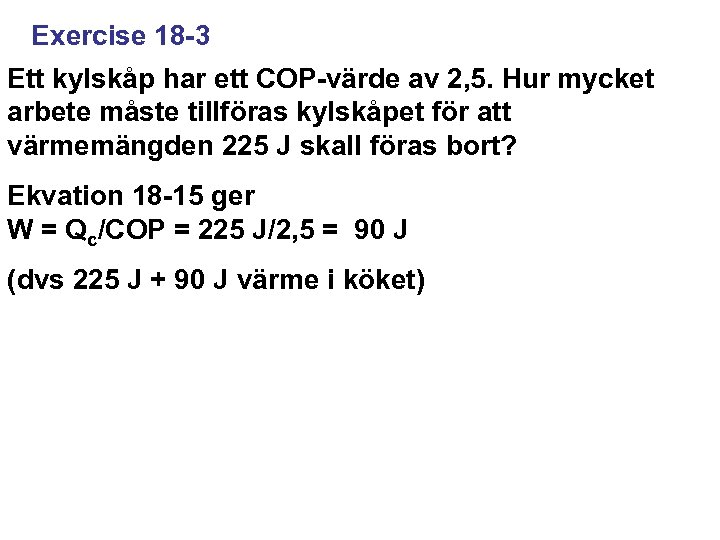Exercise 18 -3 Ett kylskåp har ett COP-värde av 2, 5. Hur mycket arbete måste tillföras kylskåpet för att värmemängden 225 J skall föras bort? Ekvation 18 -15 ger W = Qc/COP = 225 J/2, 5 = 90 J (dvs 225 J + 90 J värme i köket)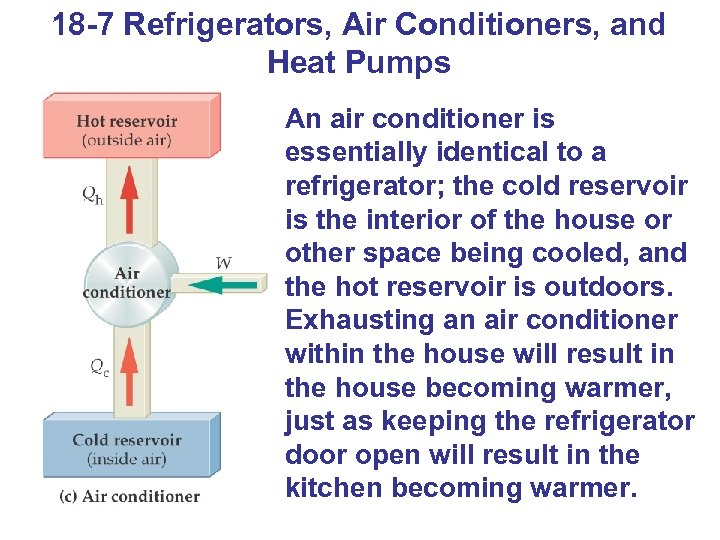18 -7 Refrigerators, Air Conditioners, and Heat Pumps An air conditioner is essentially identical to a refrigerator; the cold reservoir is the interior of the house or other space being cooled, and the hot reservoir is outdoors. Exhausting an air conditioner within the house will result in the house becoming warmer, just as keeping the refrigerator door open will result in the kitchen becoming warmer.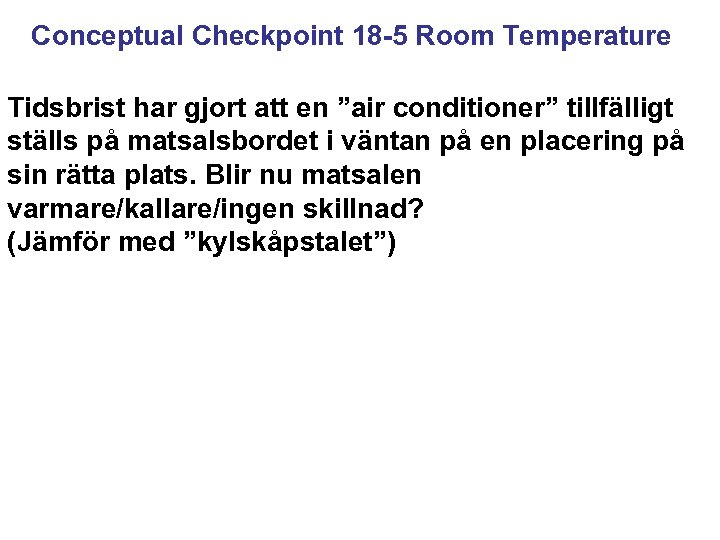Conceptual Checkpoint 18 -5 Room Temperature Tidsbrist har gjort att en ”air conditioner” tillfälligt ställs på matsalsbordet i väntan på en placering på sin rätta plats. Blir nu matsalen varmare/kallare/ingen skillnad? (Jämför med ”kylskåpstalet”)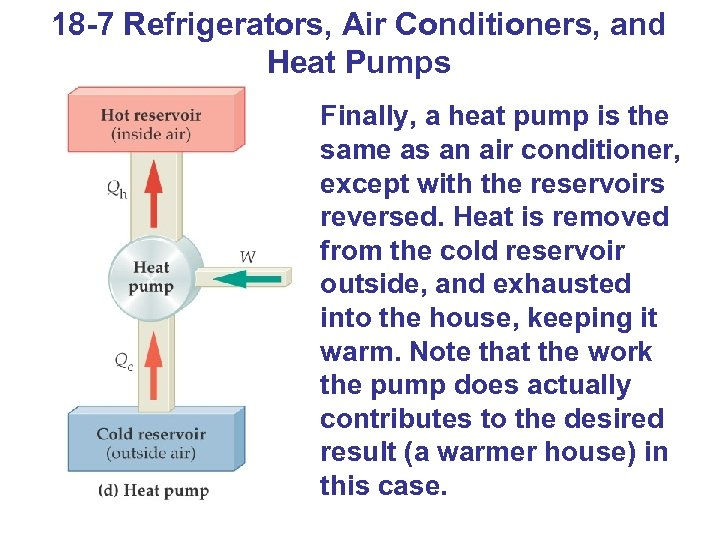18 -7 Refrigerators, Air Conditioners, and Heat Pumps Finally, a heat pump is the same as an air conditioner, except with the reservoirs reversed. Heat is removed from the cold reservoir outside, and exhausted into the house, keeping it warm. Note that the work the pump does actually contributes to the desired result (a warmer house) in this case.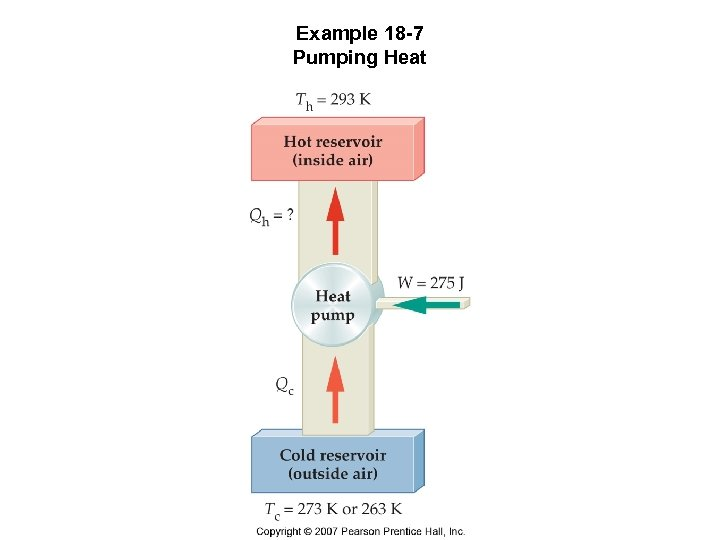Example 18 -7 Pumping Heat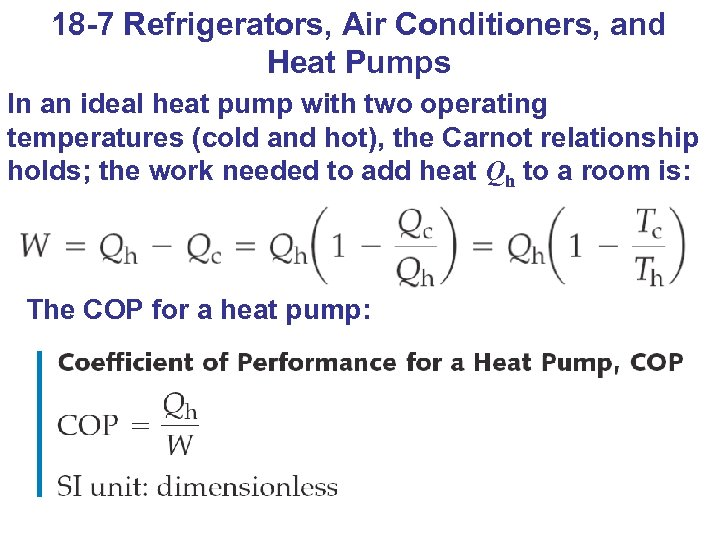18 -7 Refrigerators, Air Conditioners, and Heat Pumps In an ideal heat pump with two operating temperatures (cold and hot), the Carnot relationship holds; the work needed to add heat Qh to a room is: The COP for a heat pump: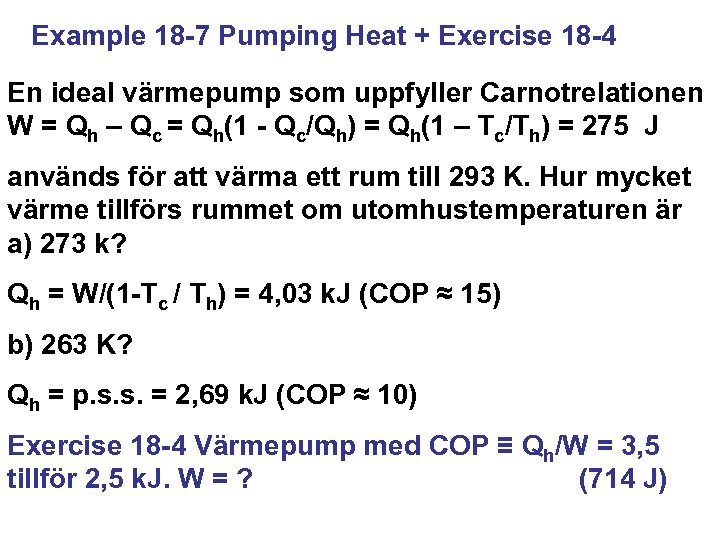Example 18 -7 Pumping Heat + Exercise 18 -4 En ideal värmepump som uppfyller Carnotrelationen W = Qh – Qc = Qh(1 - Qc/Qh) = Qh(1 – Tc/Th) = 275 J används för att värma ett rum till 293 K. Hur mycket värme tillförs rummet om utomhustemperaturen är a) 273 k? Qh = W/(1 -Tc / Th) = 4, 03 k. J (COP ≈ 15) b) 263 K? Qh = p. s. s. = 2, 69 k. J (COP ≈ 10) Exercise 18 -4 Värmepump med COP ≡ Qh/W = 3, 5 tillför 2, 5 k. J. W = ? (714 J)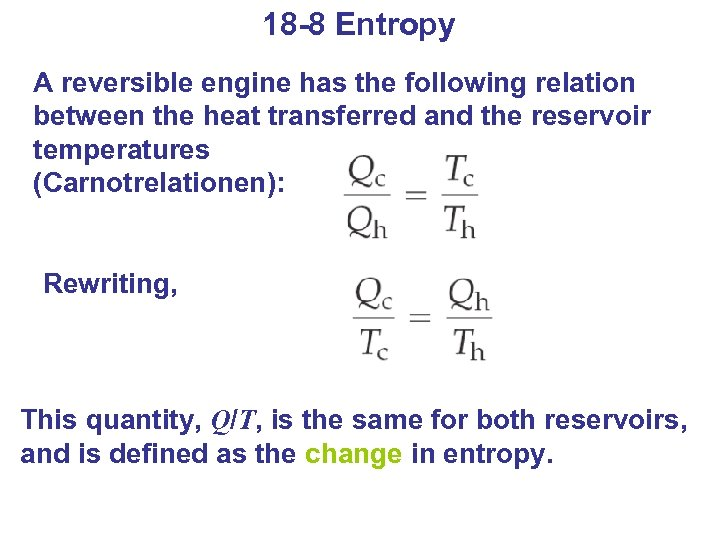18 -8 Entropy A reversible engine has the following relation between the heat transferred and the reservoir temperatures (Carnotrelationen): Rewriting, This quantity, Q/T, is the same for both reservoirs, and is defined as the change in entropy.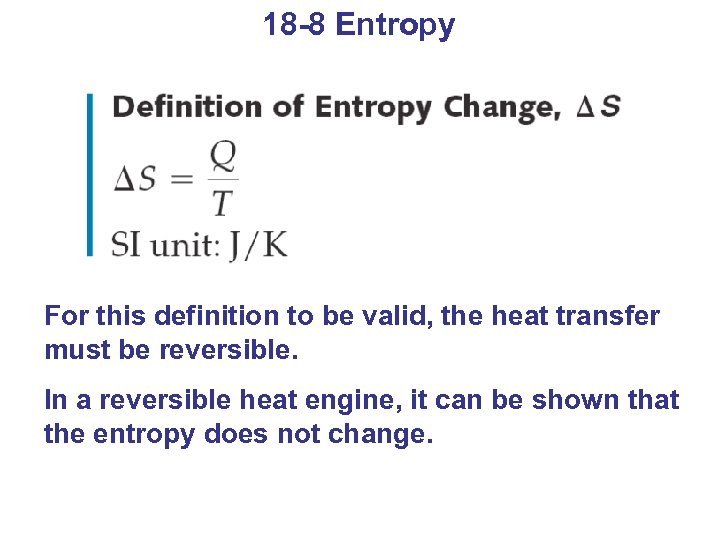18 -8 Entropy For this definition to be valid, the heat transfer must be reversible. In a reversible heat engine, it can be shown that the entropy does not change.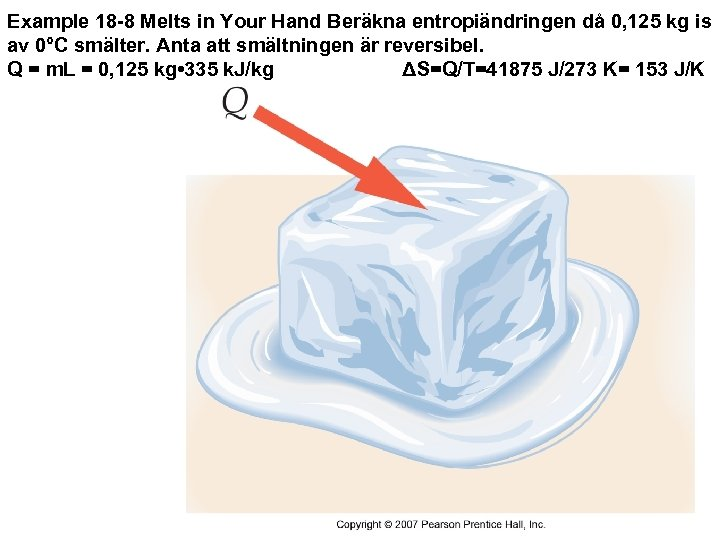Example 18 -8 Melts in Your Hand Beräkna entropiändringen då 0, 125 kg is av 0°C smälter. Anta att smältningen är reversibel. Q = m. L = 0, 125 kg • 335 k. J/kg ΔS=Q/T=41875 J/273 K= 153 J/K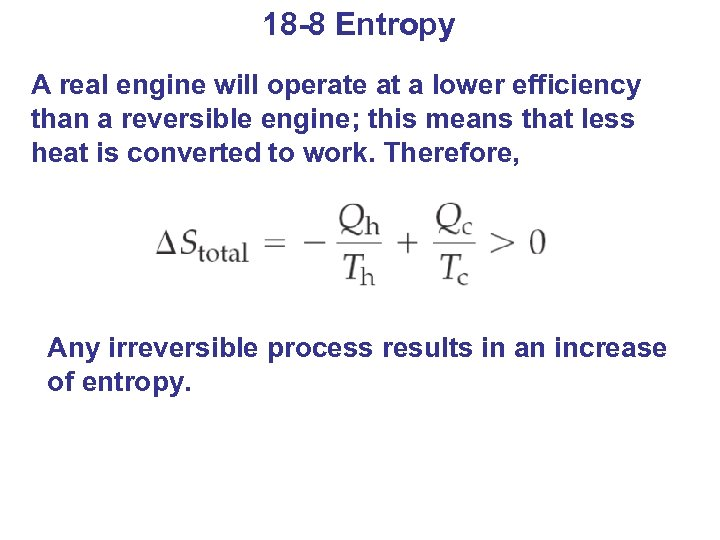18 -8 Entropy A real engine will operate at a lower efficiency than a reversible engine; this means that less heat is converted to work. Therefore, Any irreversible process results in an increase of entropy.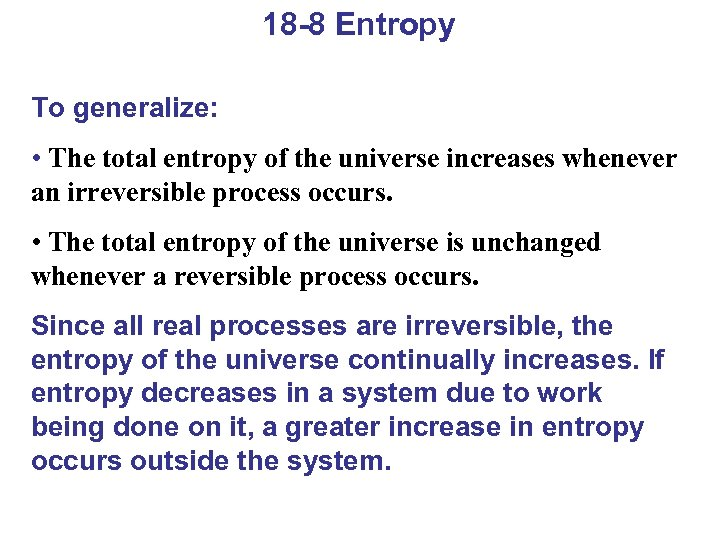18 -8 Entropy To generalize: • The total entropy of the universe increases whenever an irreversible process occurs. • The total entropy of the universe is unchanged whenever a reversible process occurs. Since all real processes are irreversible, the entropy of the universe continually increases. If entropy decreases in a system due to work being done on it, a greater increase in entropy occurs outside the system.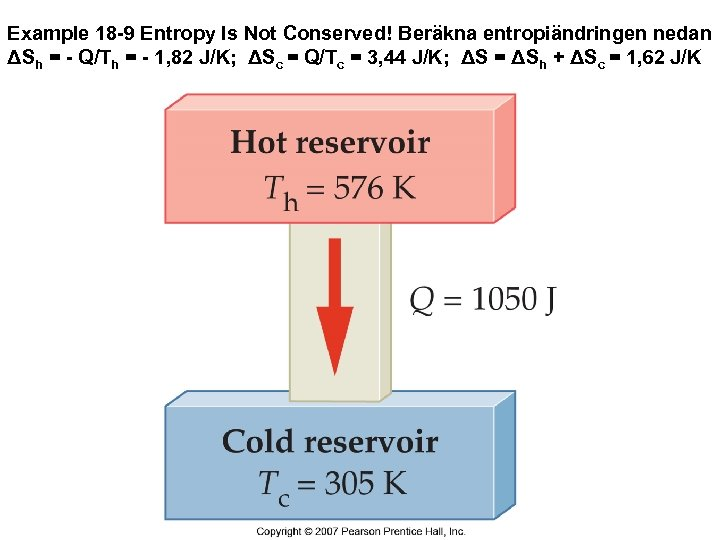Example 18 -9 Entropy Is Not Conserved! Beräkna entropiändringen nedan ΔSh = - Q/Th = - 1, 82 J/K; ΔSc = Q/Tc = 3, 44 J/K; ΔS = ΔSh + ΔSc = 1, 62 J/K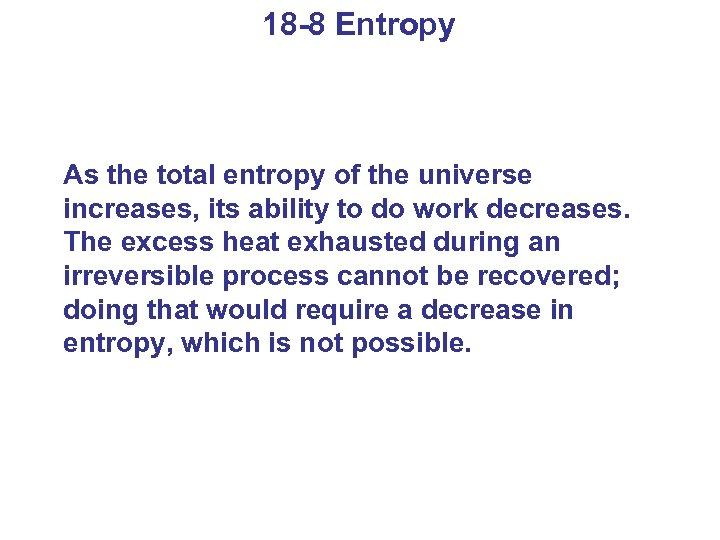18 -8 Entropy As the total entropy of the universe increases, its ability to do work decreases. The excess heat exhausted during an irreversible process cannot be recovered; doing that would require a decrease in entropy, which is not possible.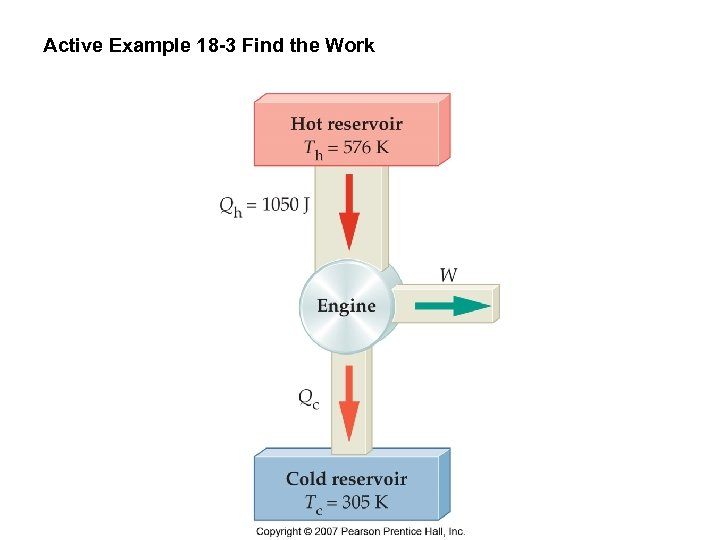Active Example 18 -3 Find the Work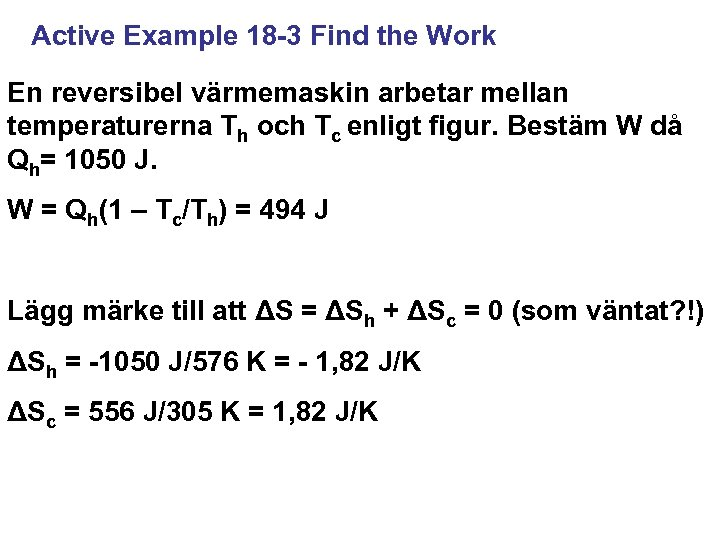Active Example 18 -3 Find the Work En reversibel värmemaskin arbetar mellan temperaturerna Th och Tc enligt figur. Bestäm W då Qh= 1050 J. W = Qh(1 – Tc/Th) = 494 J Lägg märke till att ΔS = ΔSh + ΔSc = 0 (som väntat? !) ΔSh = -1050 J/576 K = - 1, 82 J/K ΔSc = 556 J/305 K = 1, 82 J/K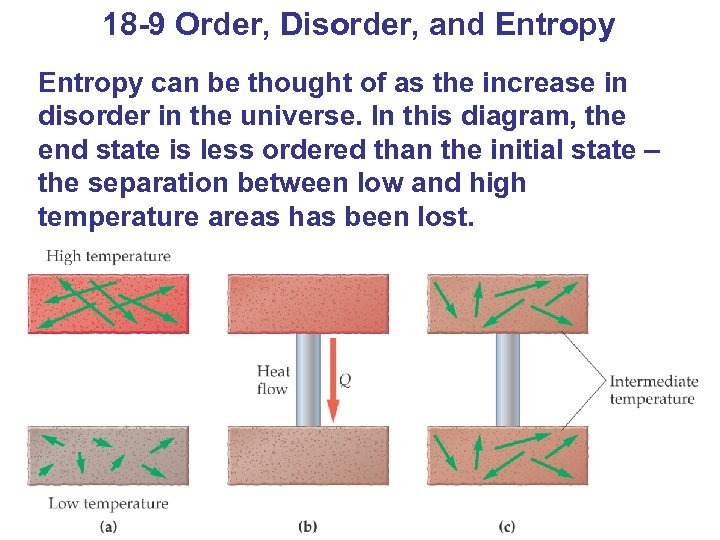18 -9 Order, Disorder, and Entropy can be thought of as the increase in disorder in the universe. In this diagram, the end state is less ordered than the initial state – the separation between low and high temperature areas has been lost.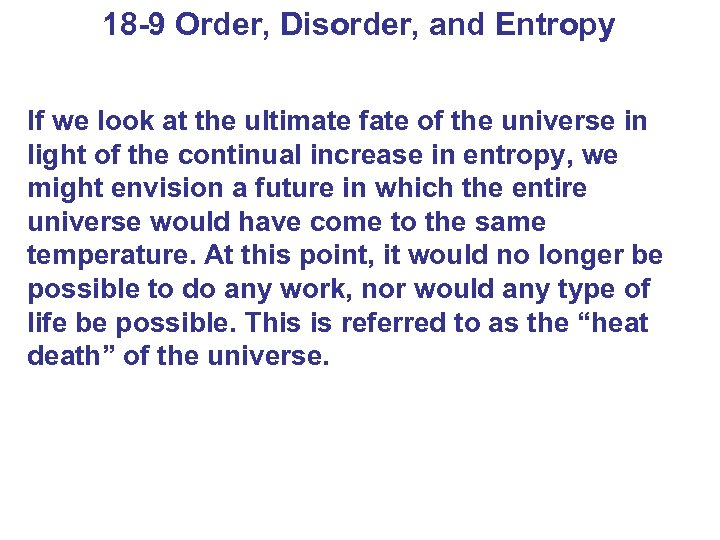18 -9 Order, Disorder, and Entropy If we look at the ultimate fate of the universe in light of the continual increase in entropy, we might envision a future in which the entire universe would have come to the same temperature. At this point, it would no longer be possible to do any work, nor would any type of life be possible. This is referred to as the “heat death” of the universe.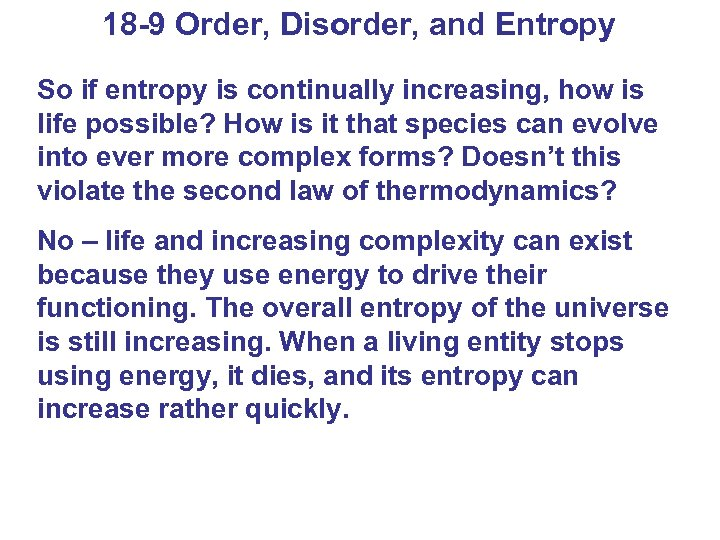18 -9 Order, Disorder, and Entropy So if entropy is continually increasing, how is life possible? How is it that species can evolve into ever more complex forms? Doesn’t this violate the second law of thermodynamics? No – life and increasing complexity can exist because they use energy to drive their functioning. The overall entropy of the universe is still increasing. When a living entity stops using energy, it dies, and its entropy can increase rather quickly.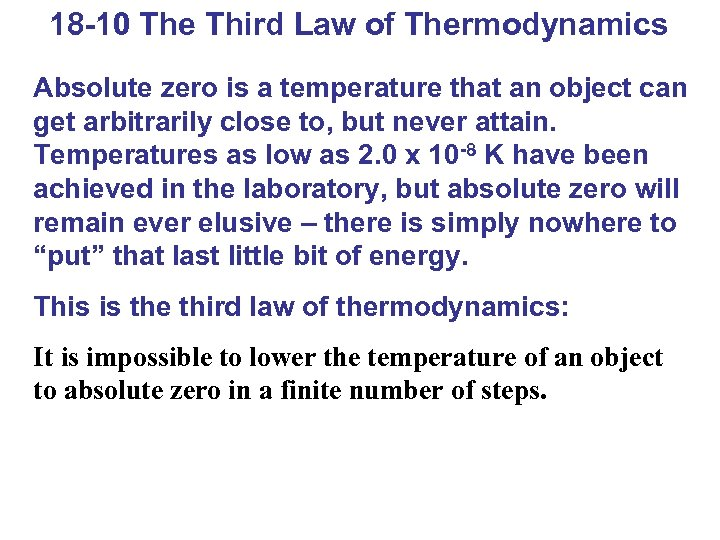18 -10 The Third Law of Thermodynamics Absolute zero is a temperature that an object can get arbitrarily close to, but never attain. Temperatures as low as 2. 0 x 10 -8 K have been achieved in the laboratory, but absolute zero will remain ever elusive – there is simply nowhere to “put” that last little bit of energy. This is the third law of thermodynamics: It is impossible to lower the temperature of an object to absolute zero in a finite number of steps.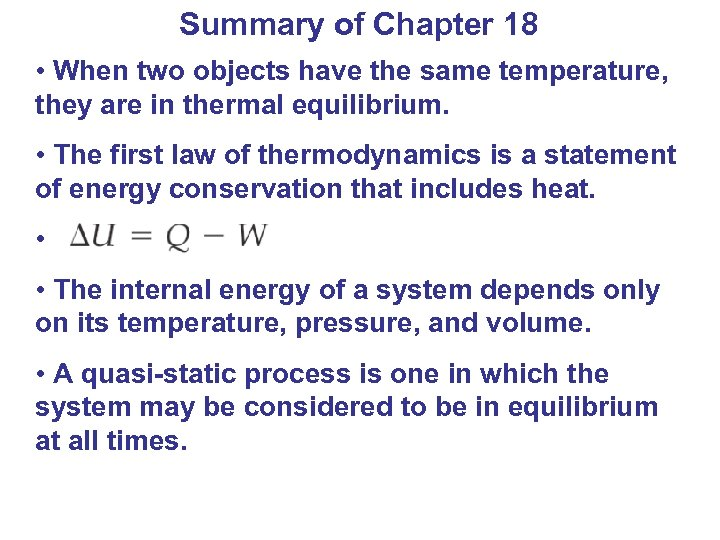Summary of Chapter 18 • When two objects have the same temperature, they are in thermal equilibrium. • The first law of thermodynamics is a statement of energy conservation that includes heat. • • The internal energy of a system depends only on its temperature, pressure, and volume. • A quasi-static process is one in which the system may be considered to be in equilibrium at all times.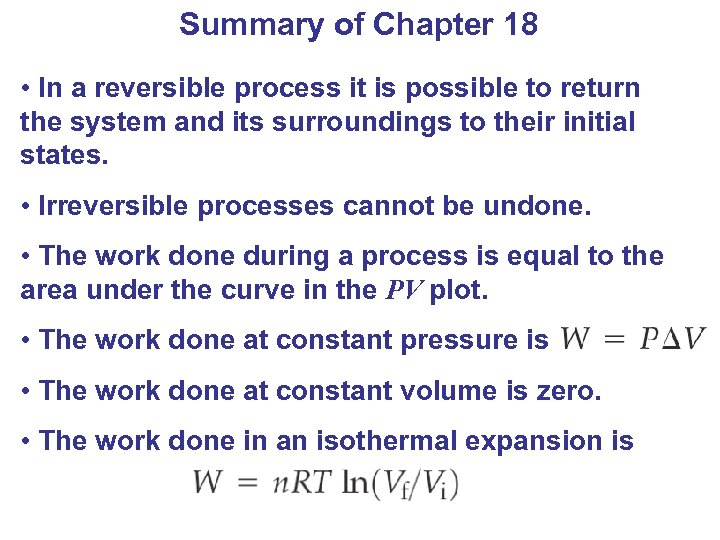Summary of Chapter 18 • In a reversible process it is possible to return the system and its surroundings to their initial states. • Irreversible processes cannot be undone. • The work done during a process is equal to the area under the curve in the PV plot. • The work done at constant pressure is • The work done at constant volume is zero. • The work done in an isothermal expansion is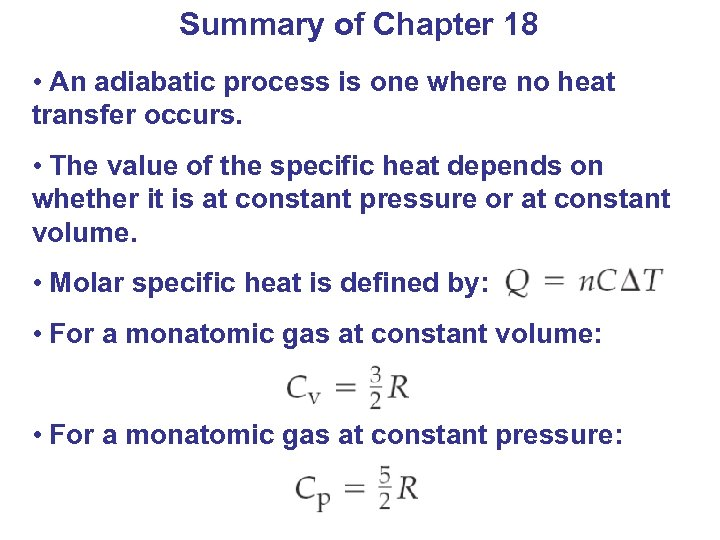Summary of Chapter 18 • An adiabatic process is one where no heat transfer occurs. • The value of the specific heat depends on whether it is at constant pressure or at constant volume. • Molar specific heat is defined by: • For a monatomic gas at constant volume: • For a monatomic gas at constant pressure: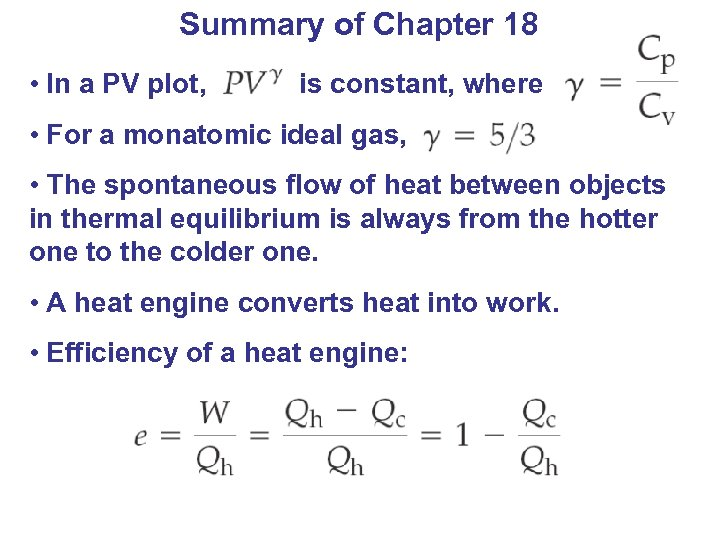Summary of Chapter 18 • In a PV plot, is constant, where • For a monatomic ideal gas, • The spontaneous flow of heat between objects in thermal equilibrium is always from the hotter one to the colder one. • A heat engine converts heat into work. • Efficiency of a heat engine: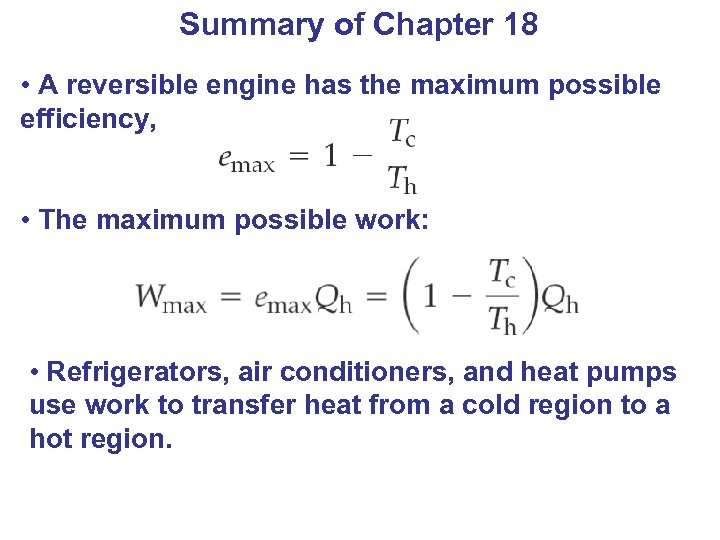Summary of Chapter 18 • A reversible engine has the maximum possible efficiency, • The maximum possible work: • Refrigerators, air conditioners, and heat pumps use work to transfer heat from a cold region to a hot region.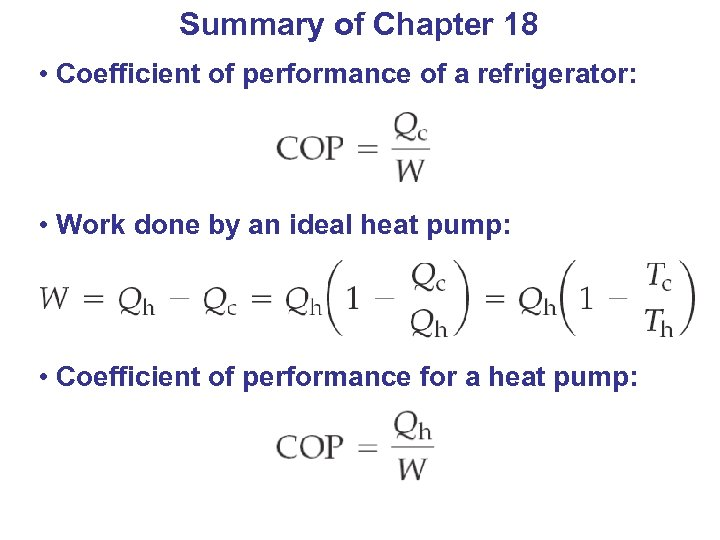Summary of Chapter 18 • Coefficient of performance of a refrigerator: • Work done by an ideal heat pump: • Coefficient of performance for a heat pump: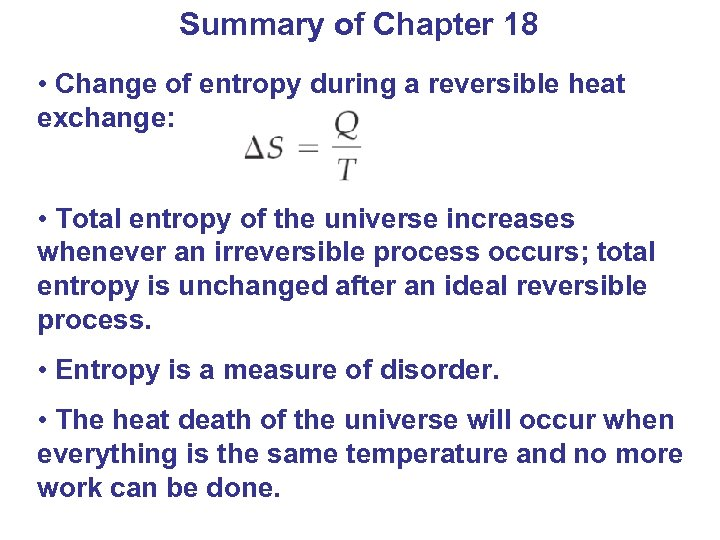Summary of Chapter 18 • Change of entropy during a reversible heat exchange: • Total entropy of the universe increases whenever an irreversible process occurs; total entropy is unchanged after an ideal reversible process. • Entropy is a measure of disorder. • The heat death of the universe will occur when everything is the same temperature and no more work can be done.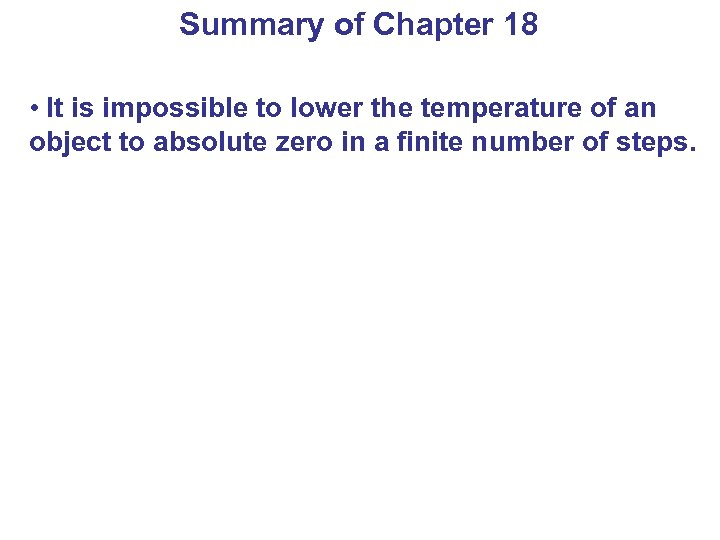Summary of Chapter 18 • It is impossible to lower the temperature of an object to absolute zero in a finite number of steps.